# Intermediate maths solutions for Theory of Equations

Intermediate mathematics IIA chapter 4 Theory of Equations exercises 4(a), 4(b), 4(c) and 4(d) (text book) solutions are given.

These solutions are very easy to understand.

First study the text book lessons Theory of Equations very well.

Then observe the given solutions and try them in your own method.

You can also see

Inter Maths 1A textbook solutions

Inter Maths 1B textbook solutions

Inter Maths IIA textbook solutions

Inter Maths IIB textbook solutions

M

# Theory of Equations solutions inter

Exercise 4(a)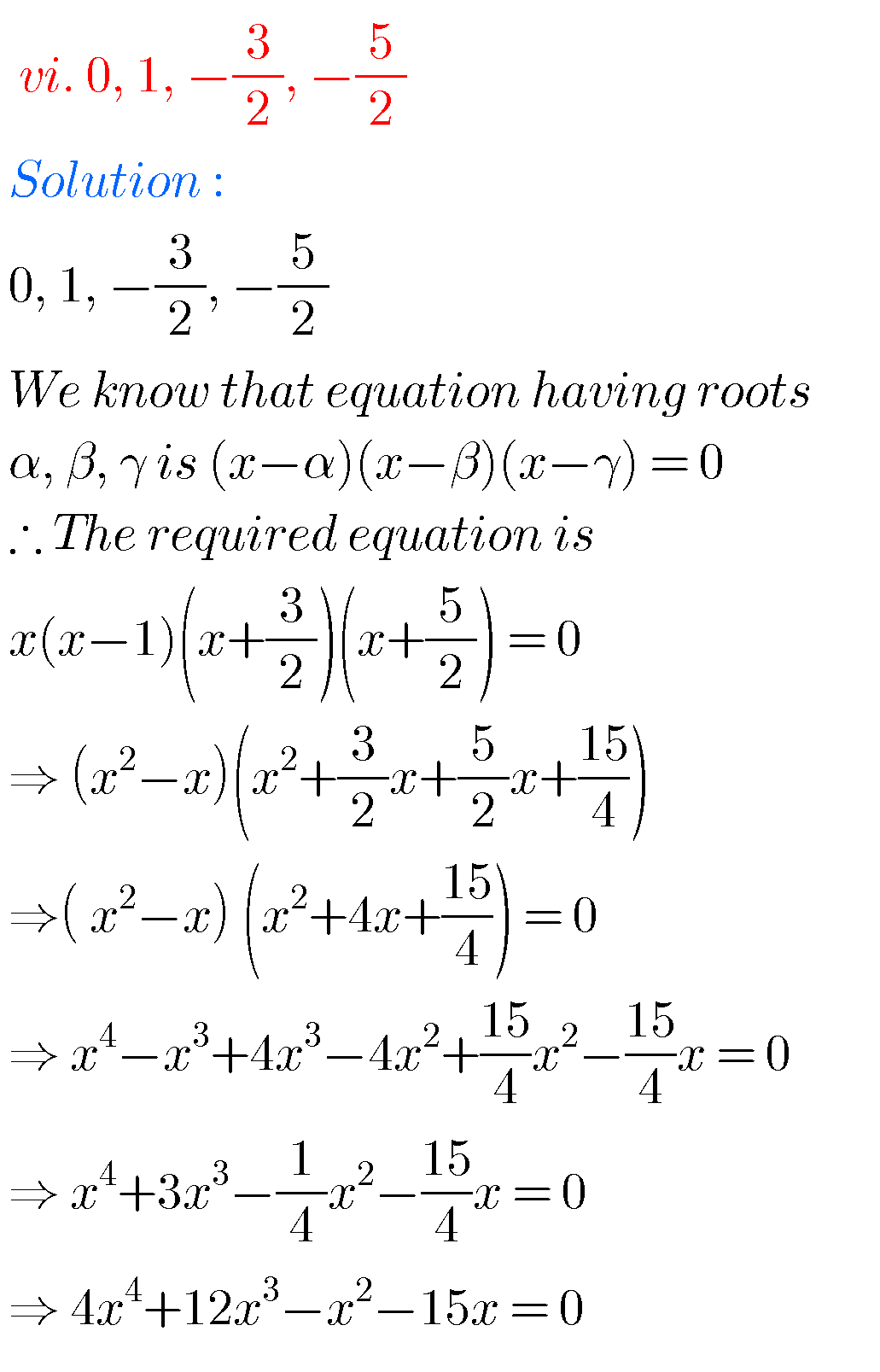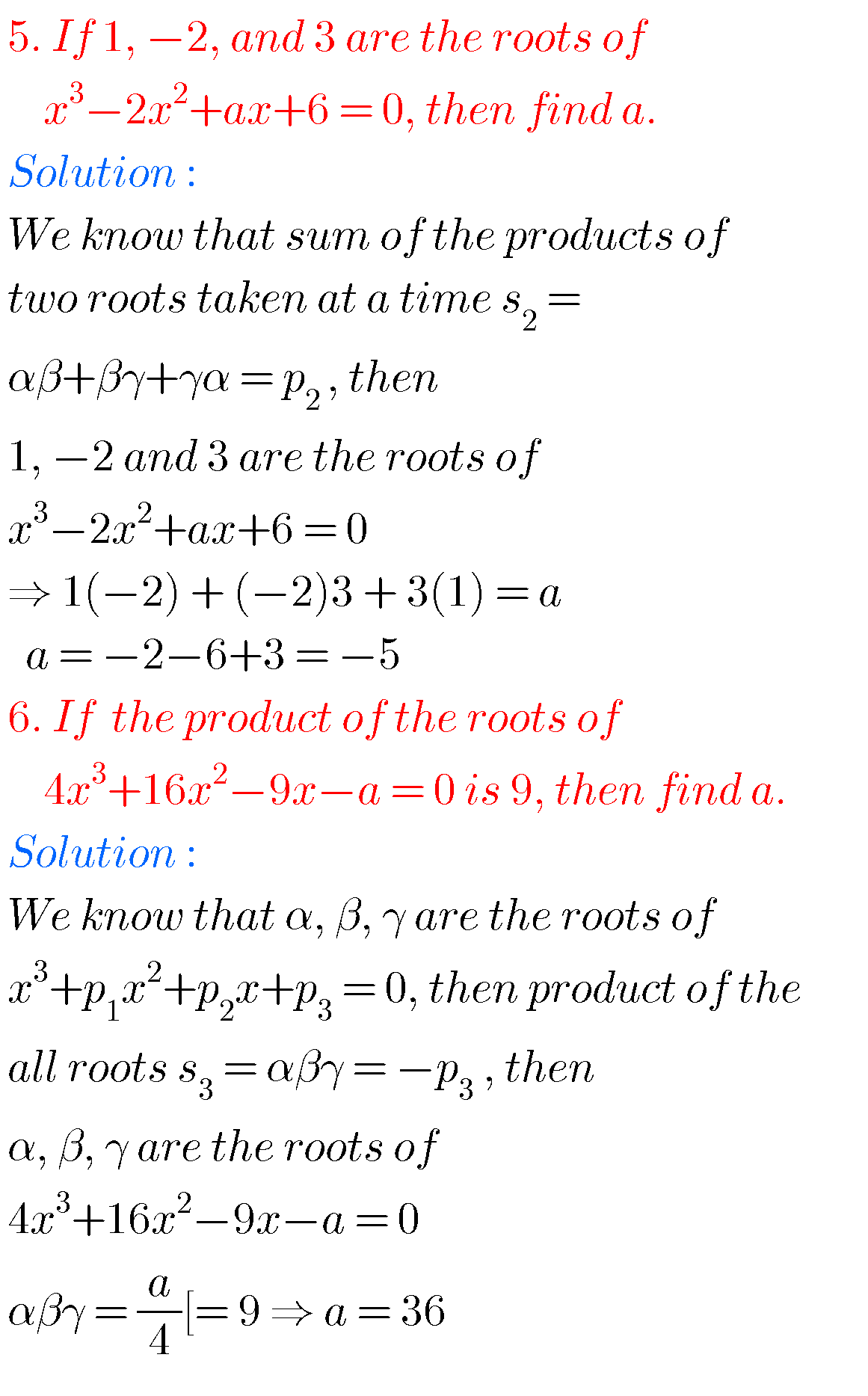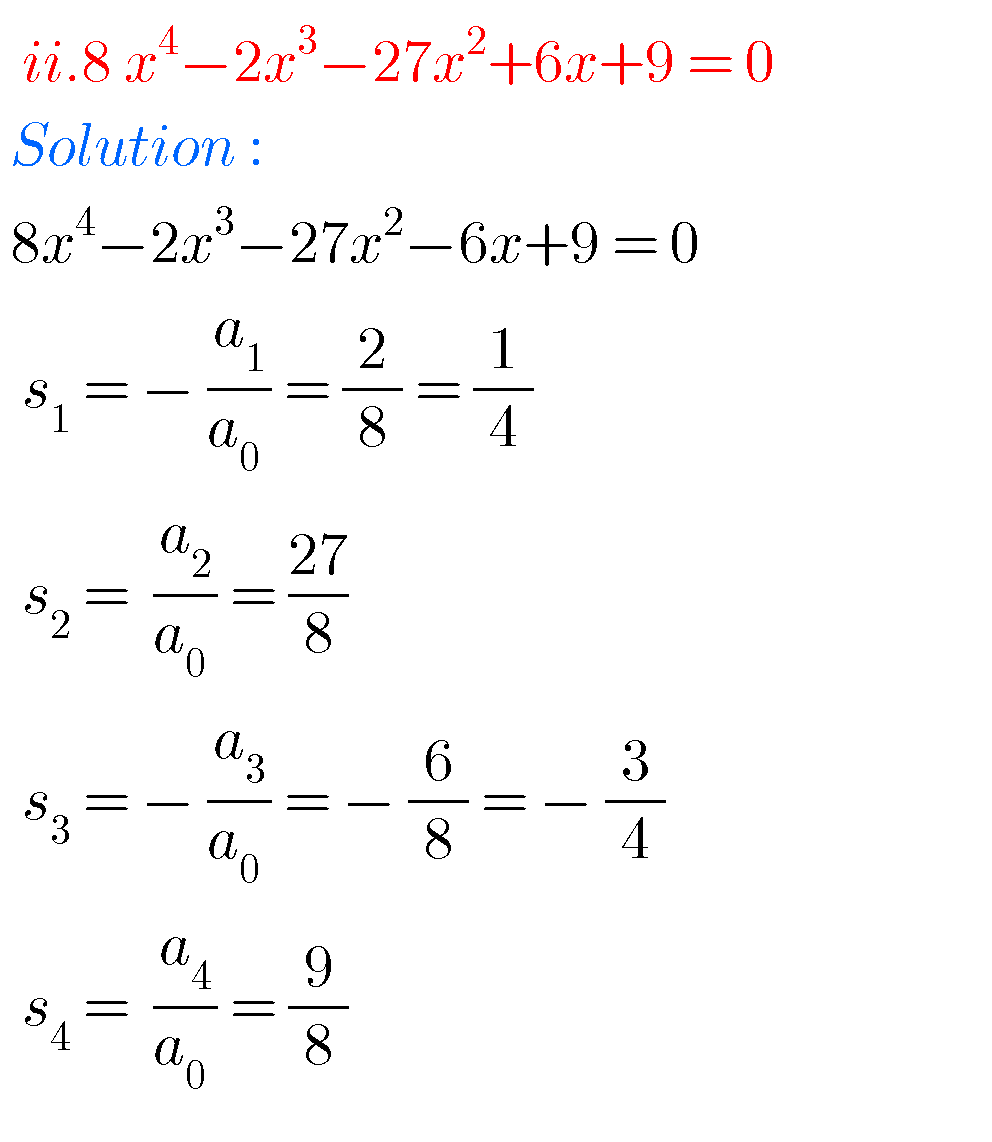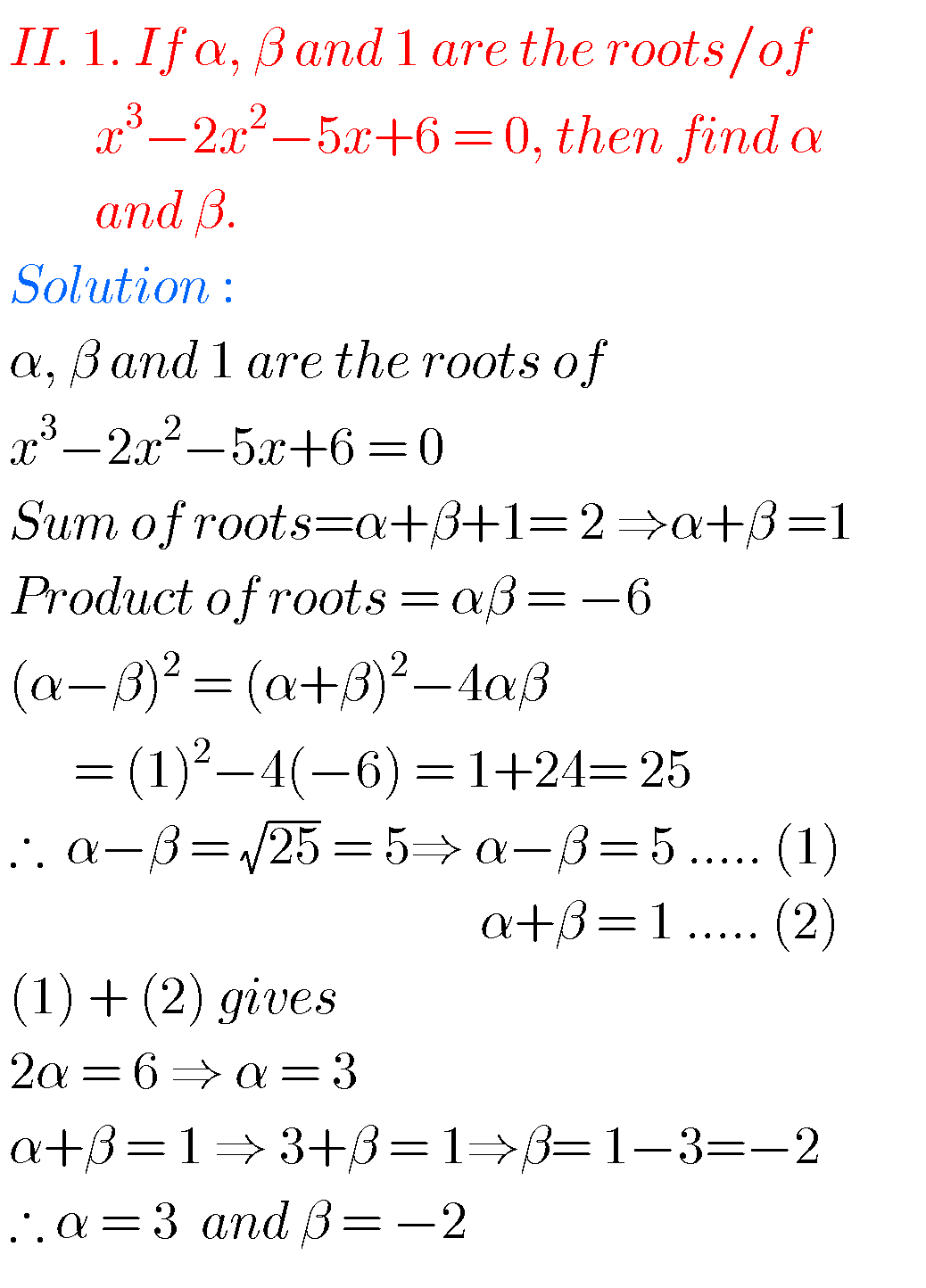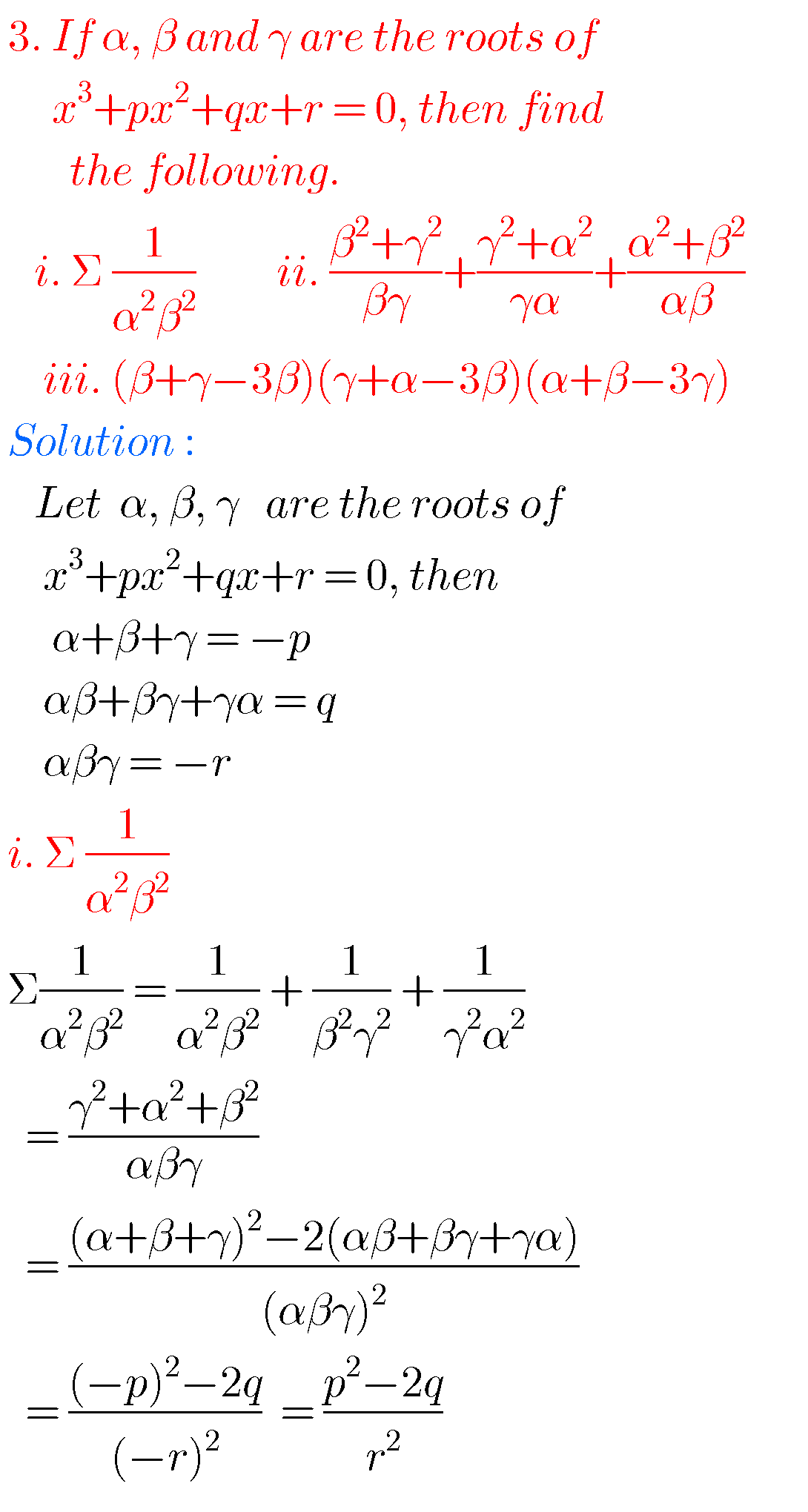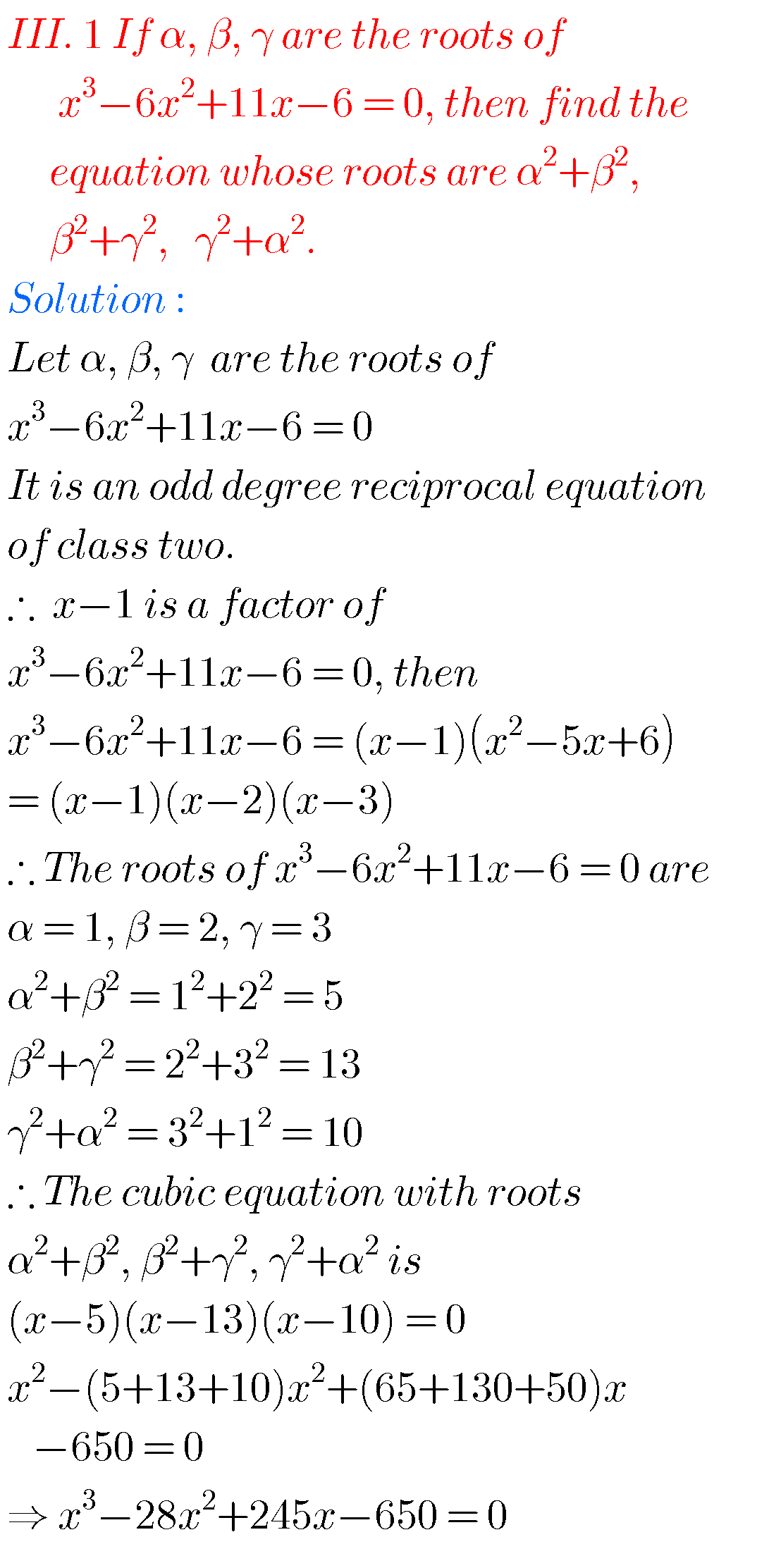## Textual exercises solutions for theory of equations inter

Exercise 4(b)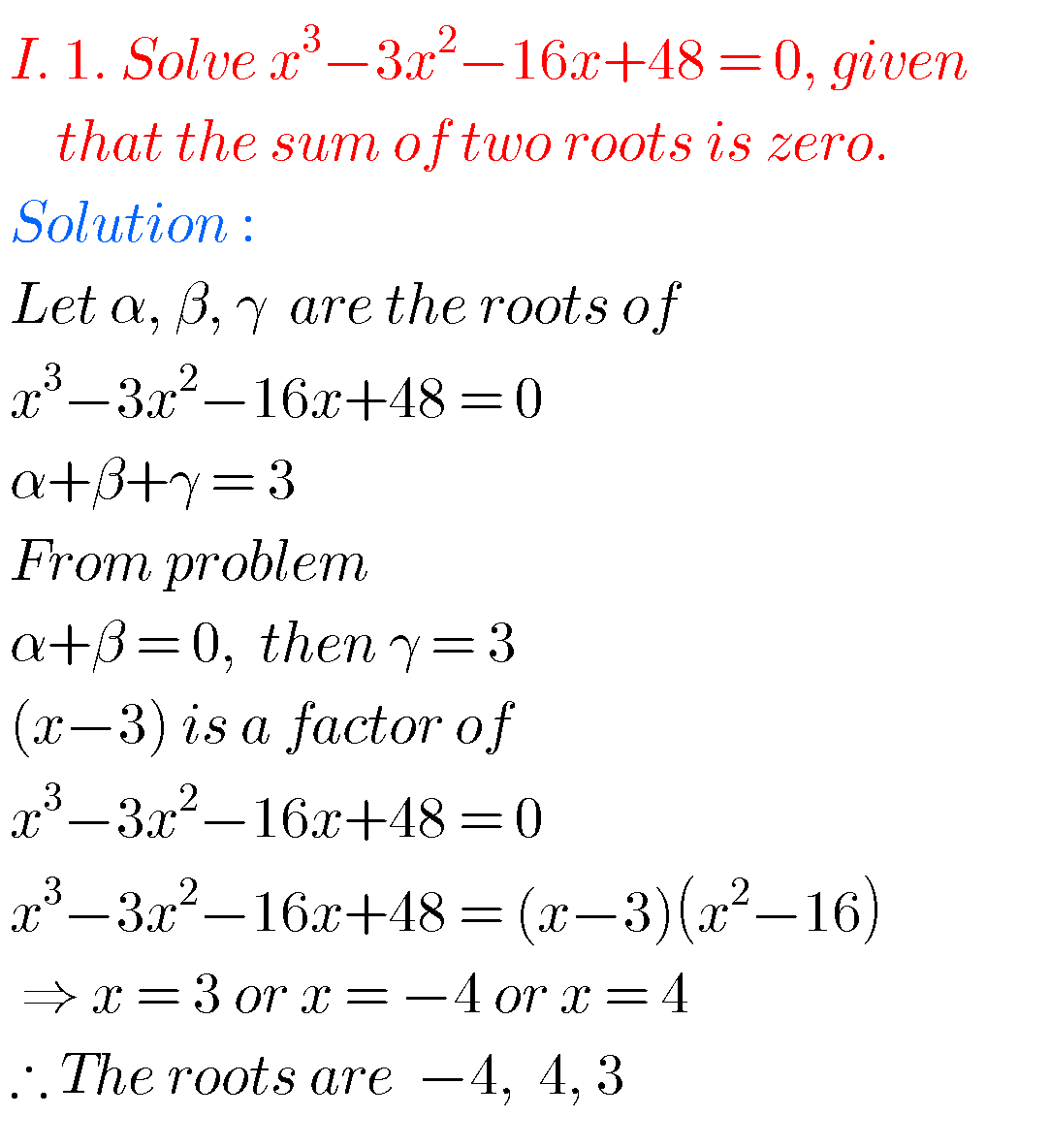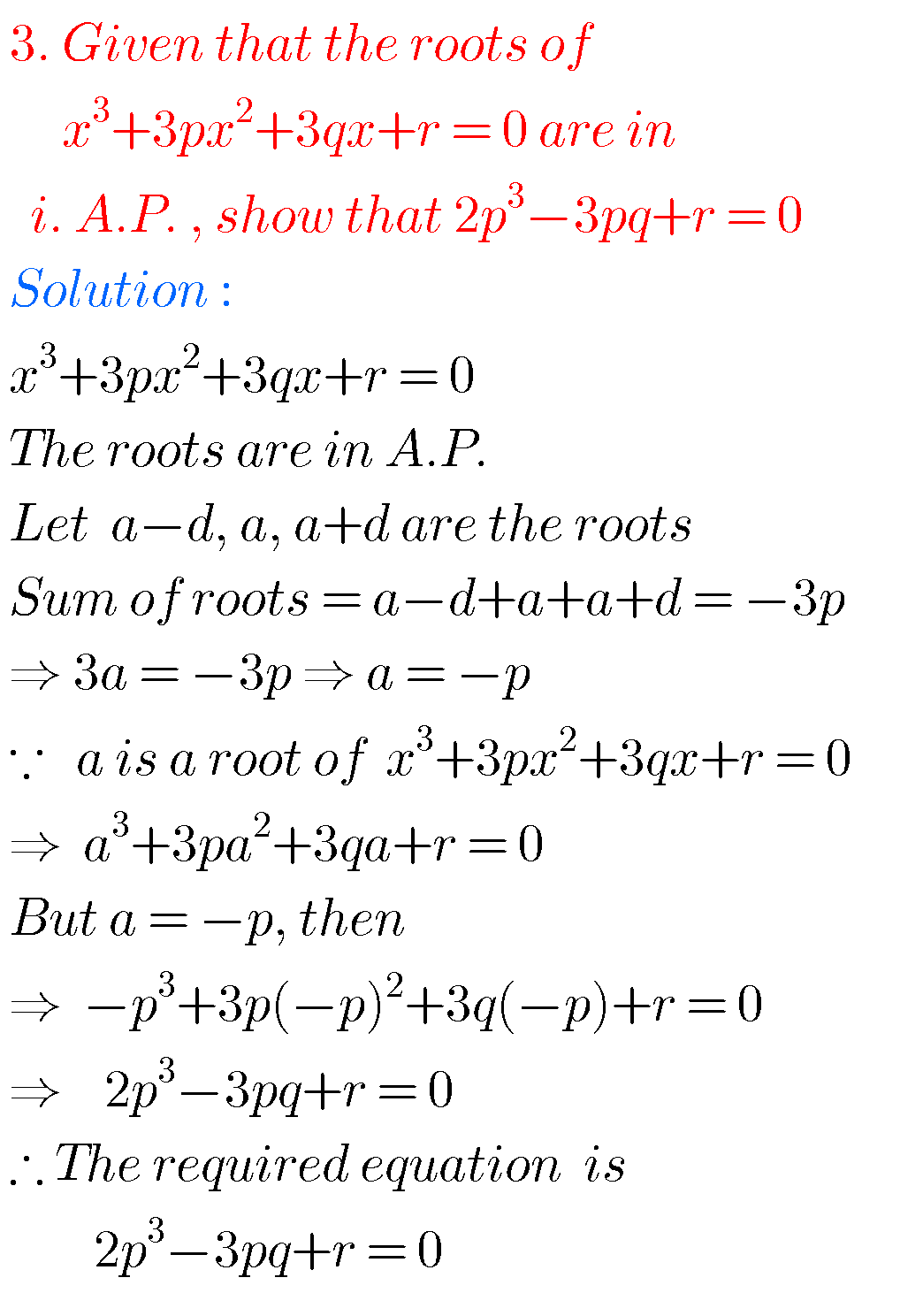##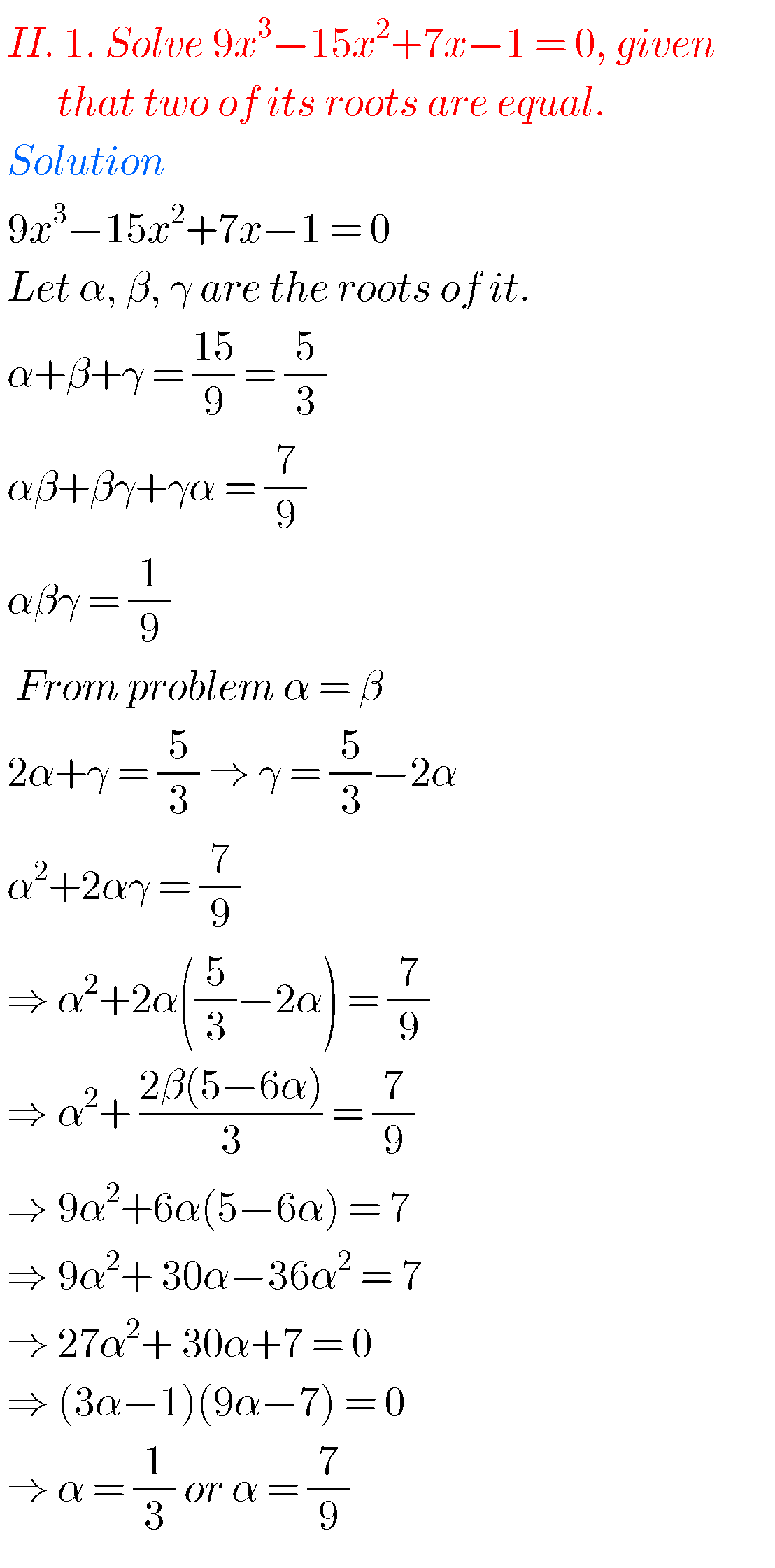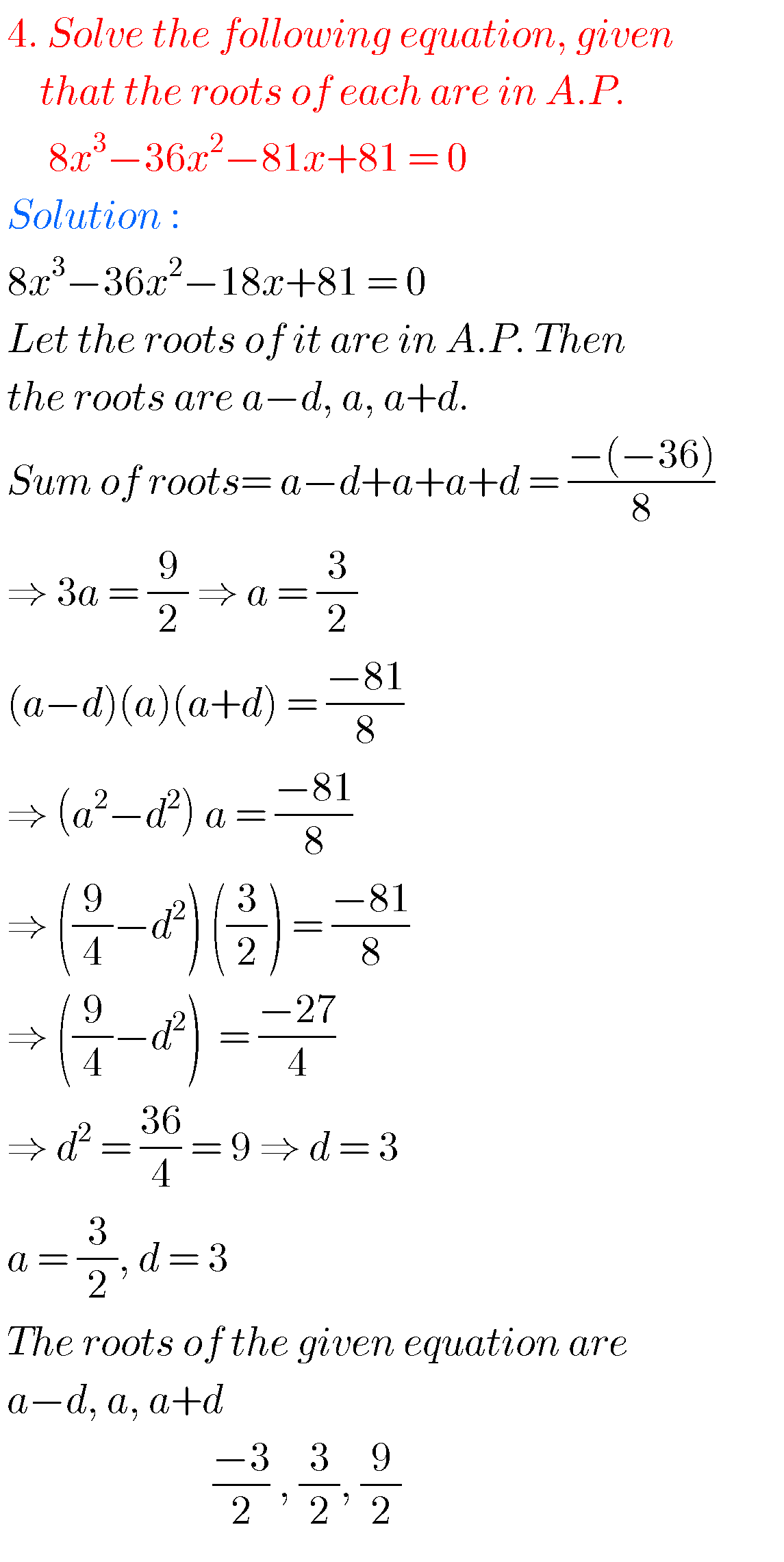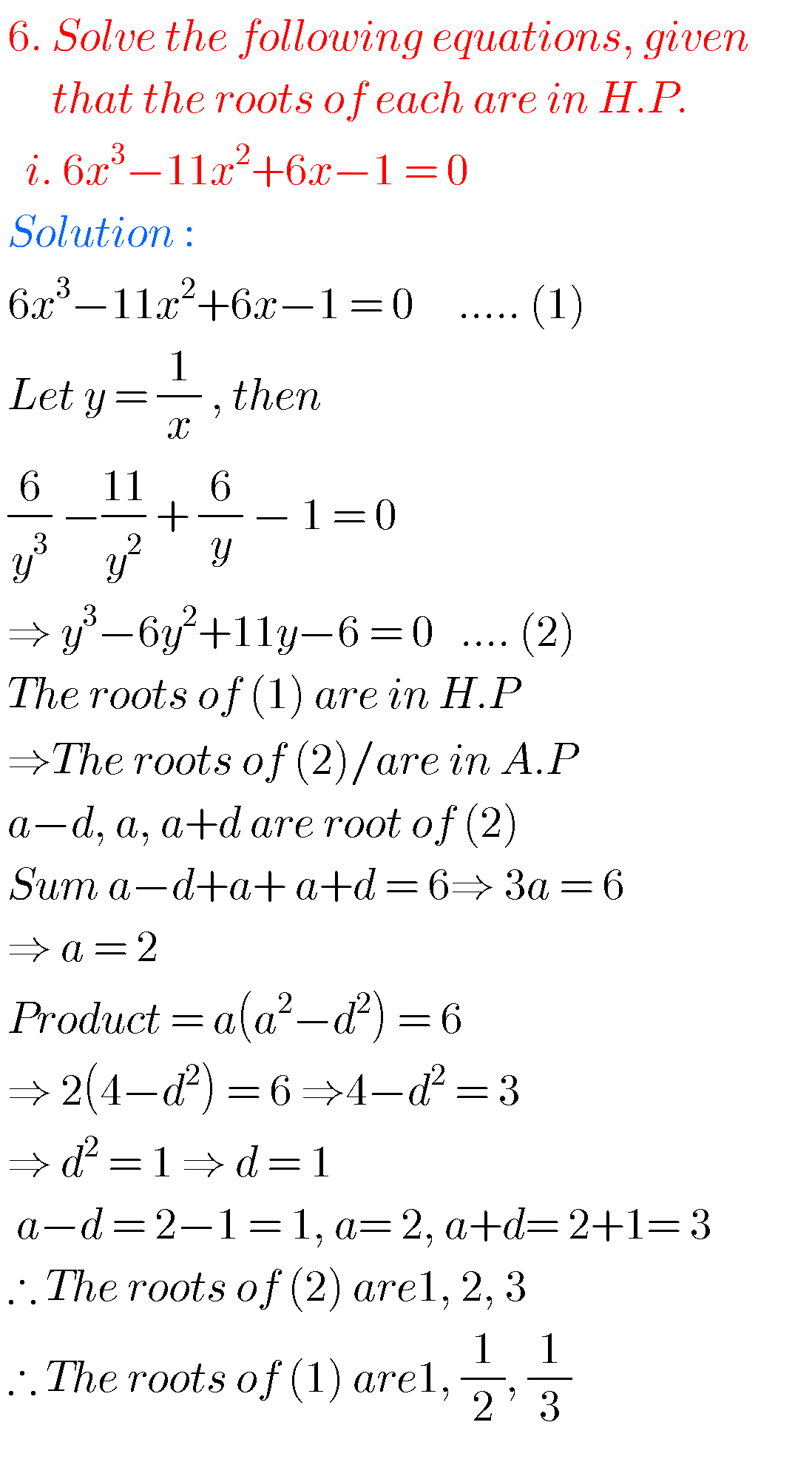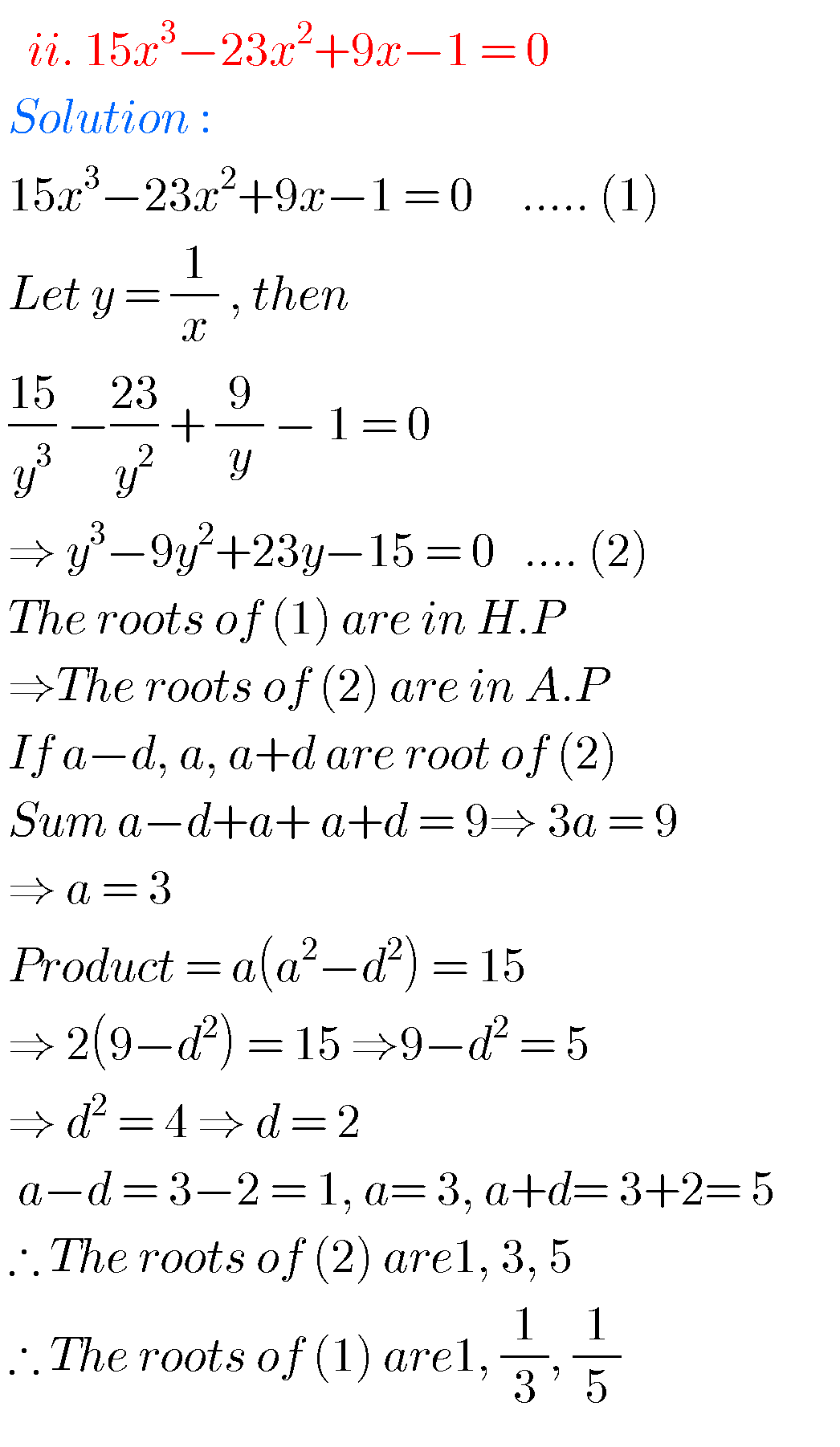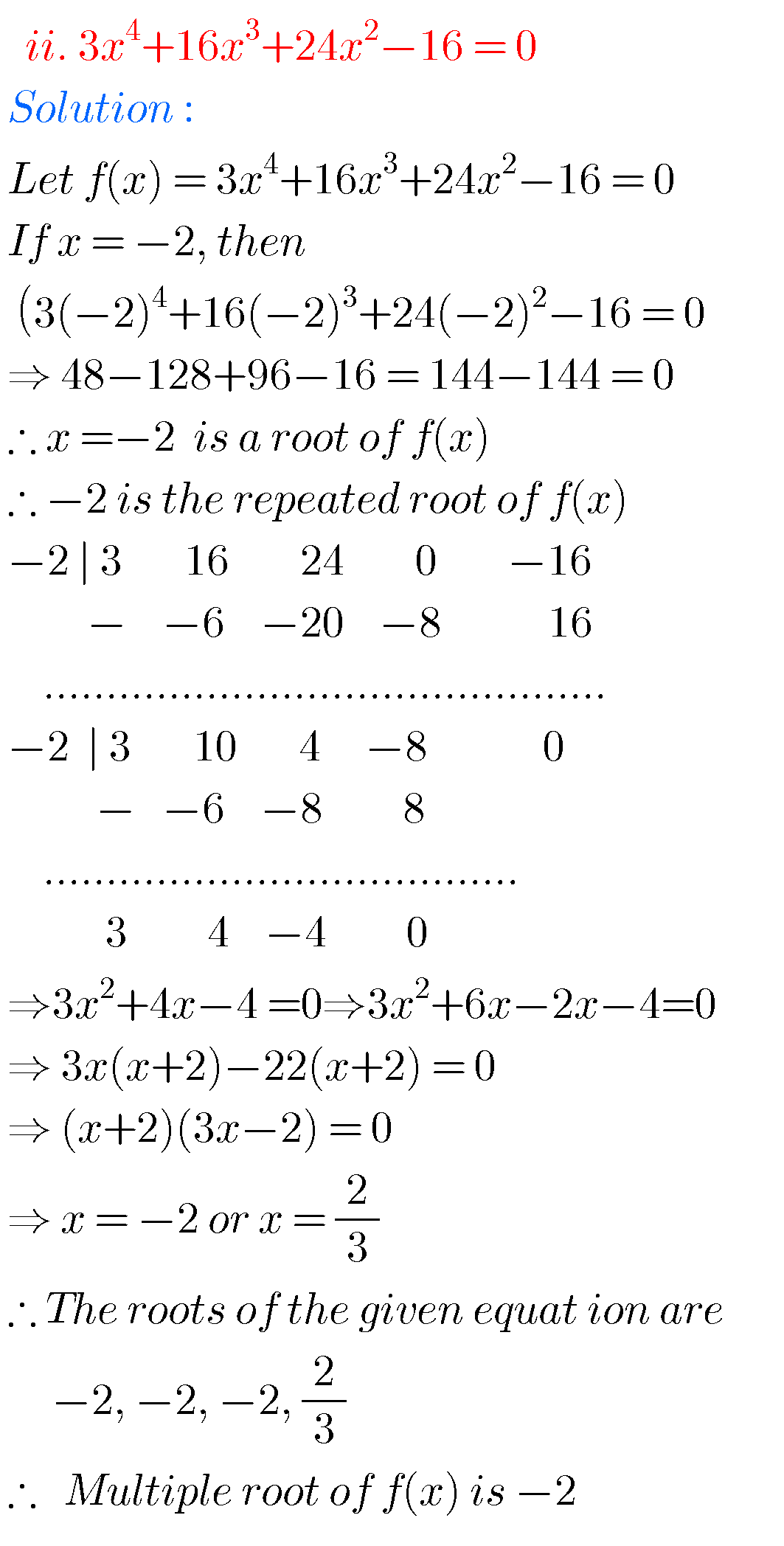#### Solutions for theory of equations inter maths

Exercise 4(c)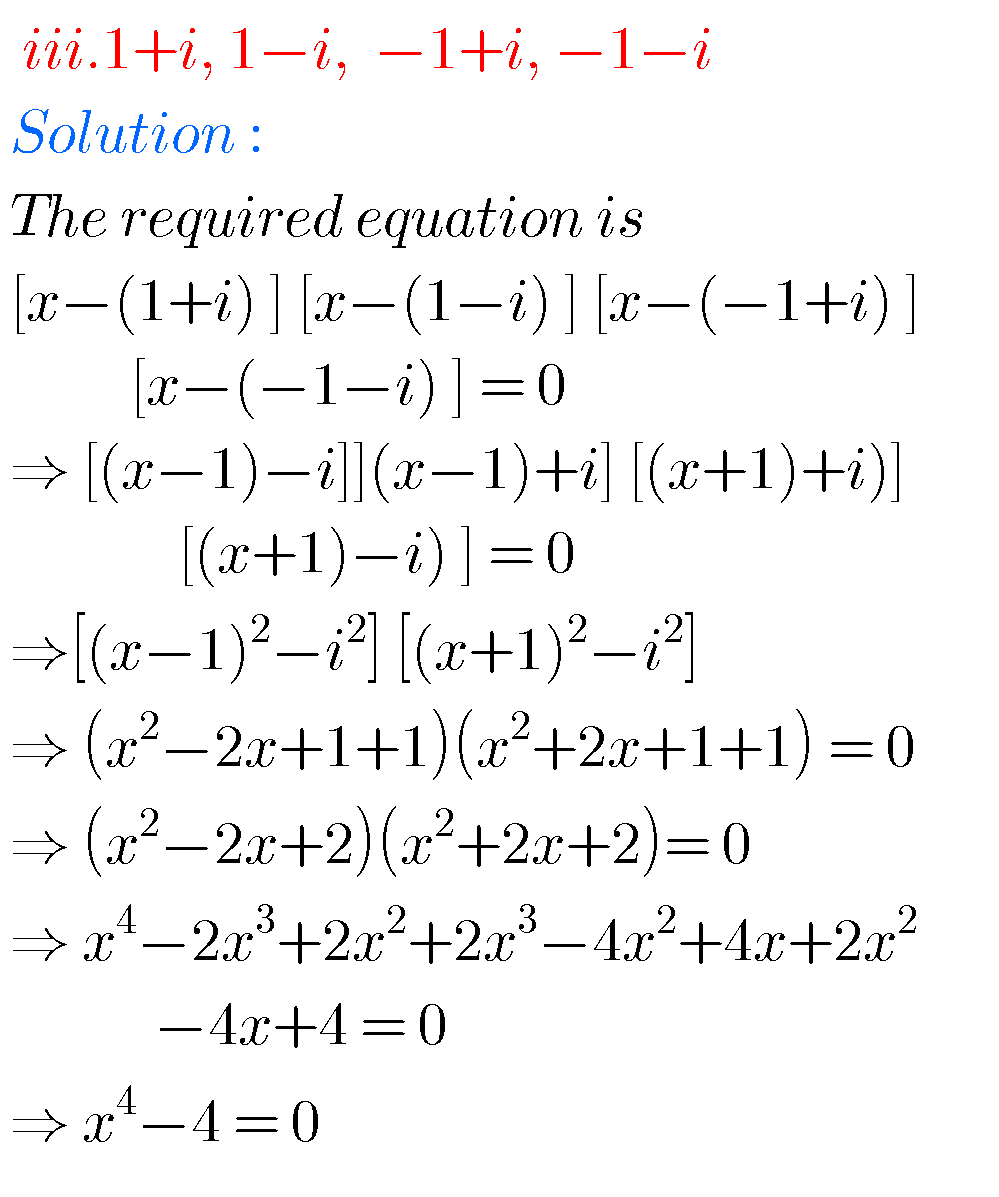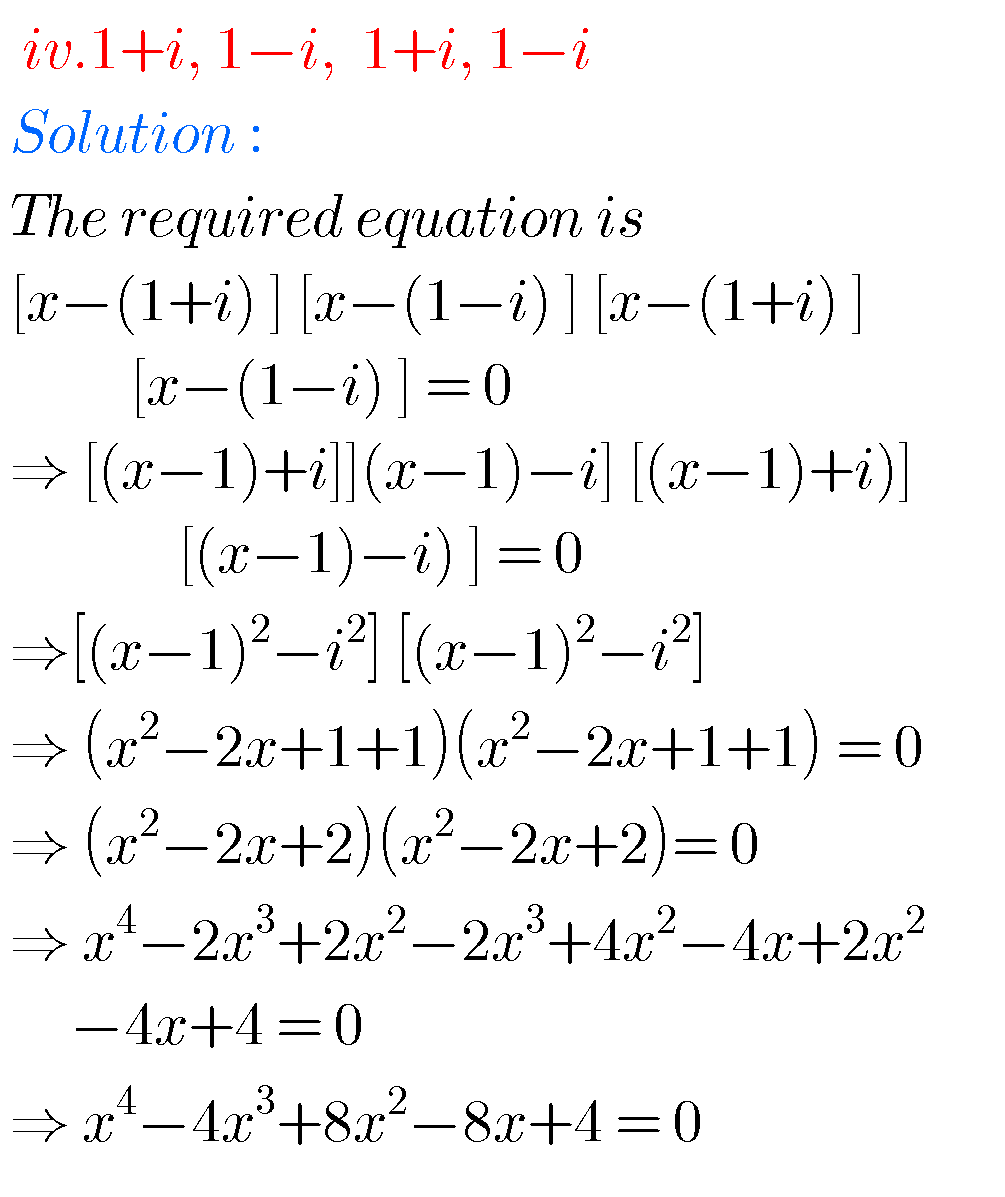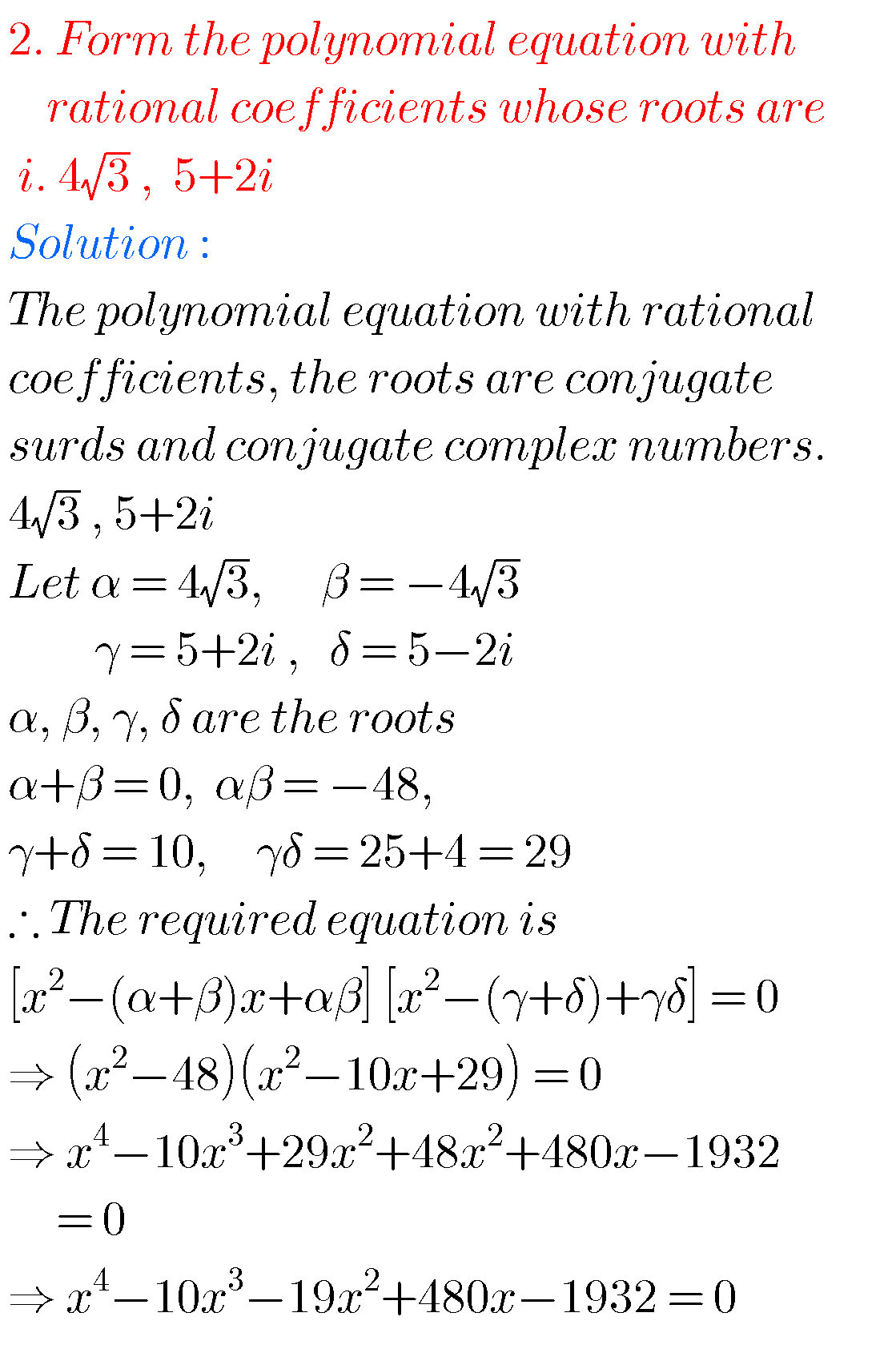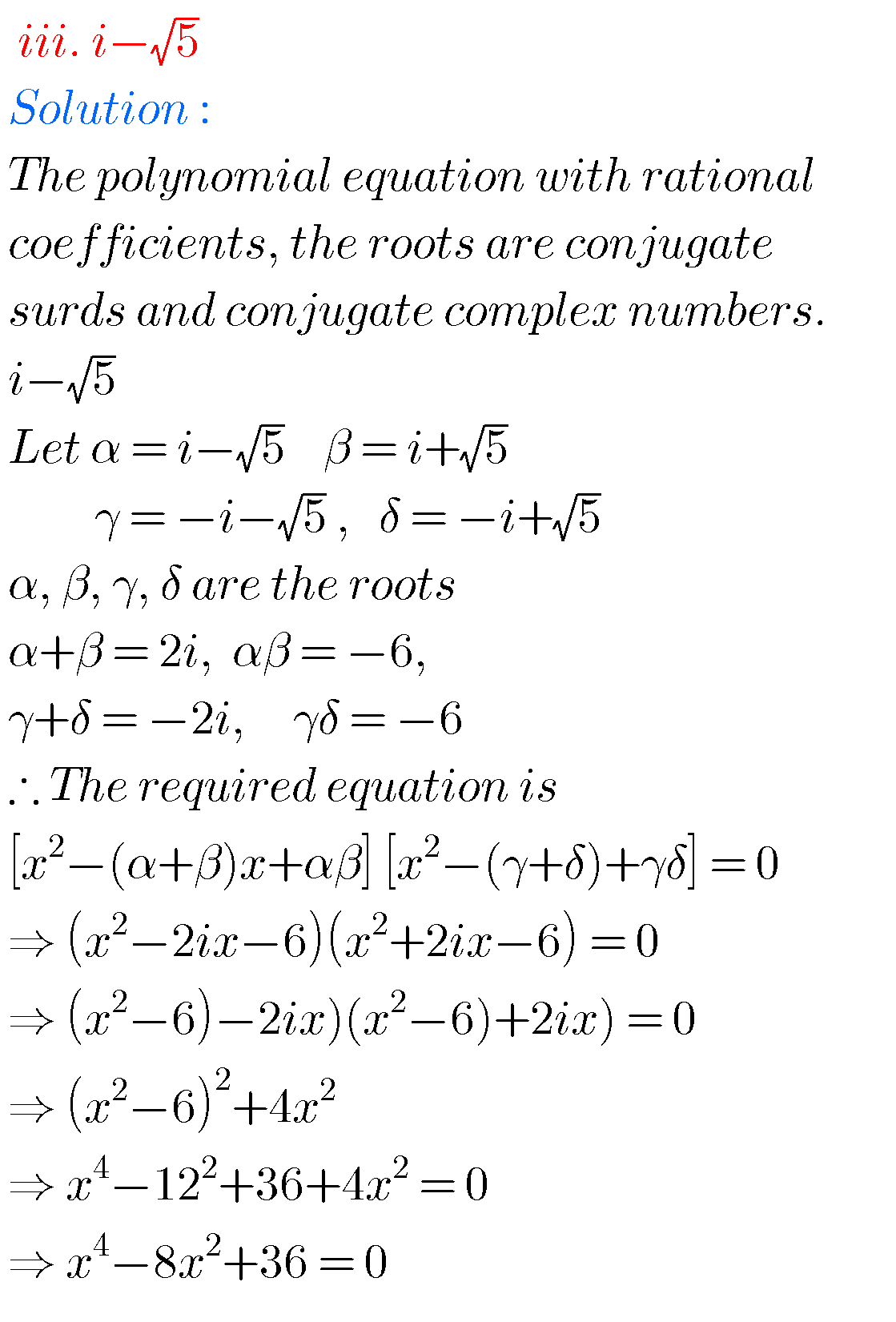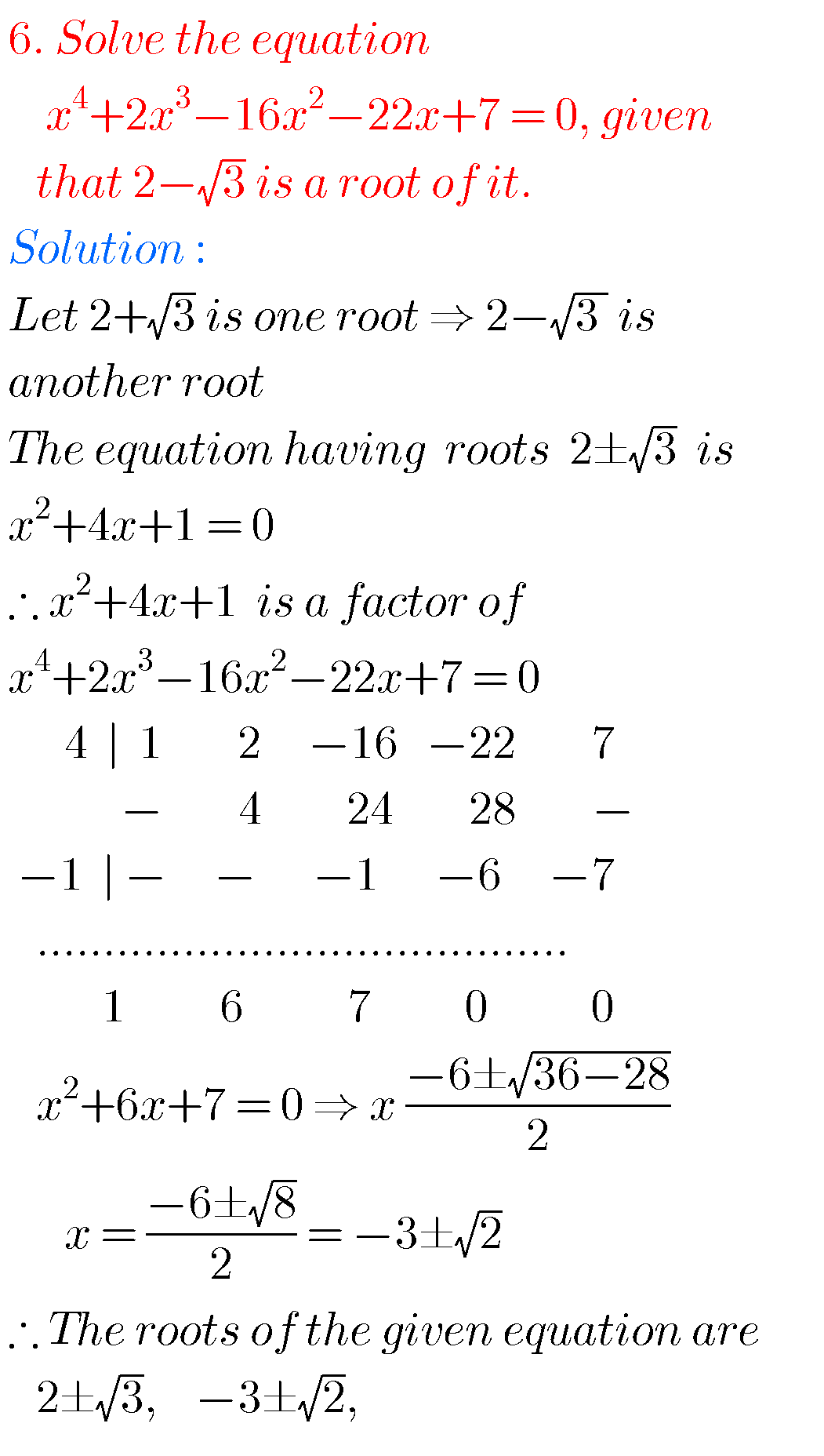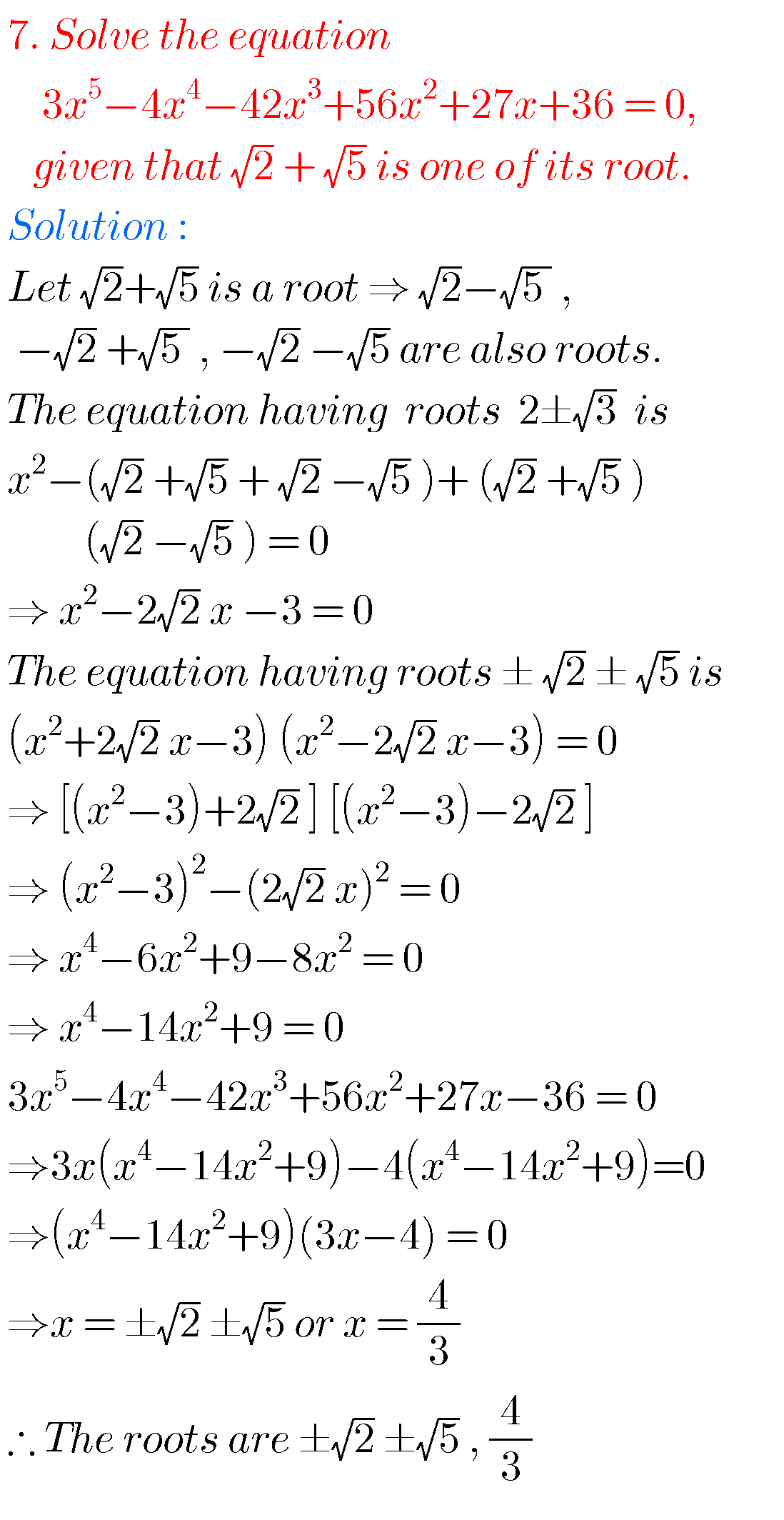### Maths IIA inter solutions for chapter 4

Exercise 4(d)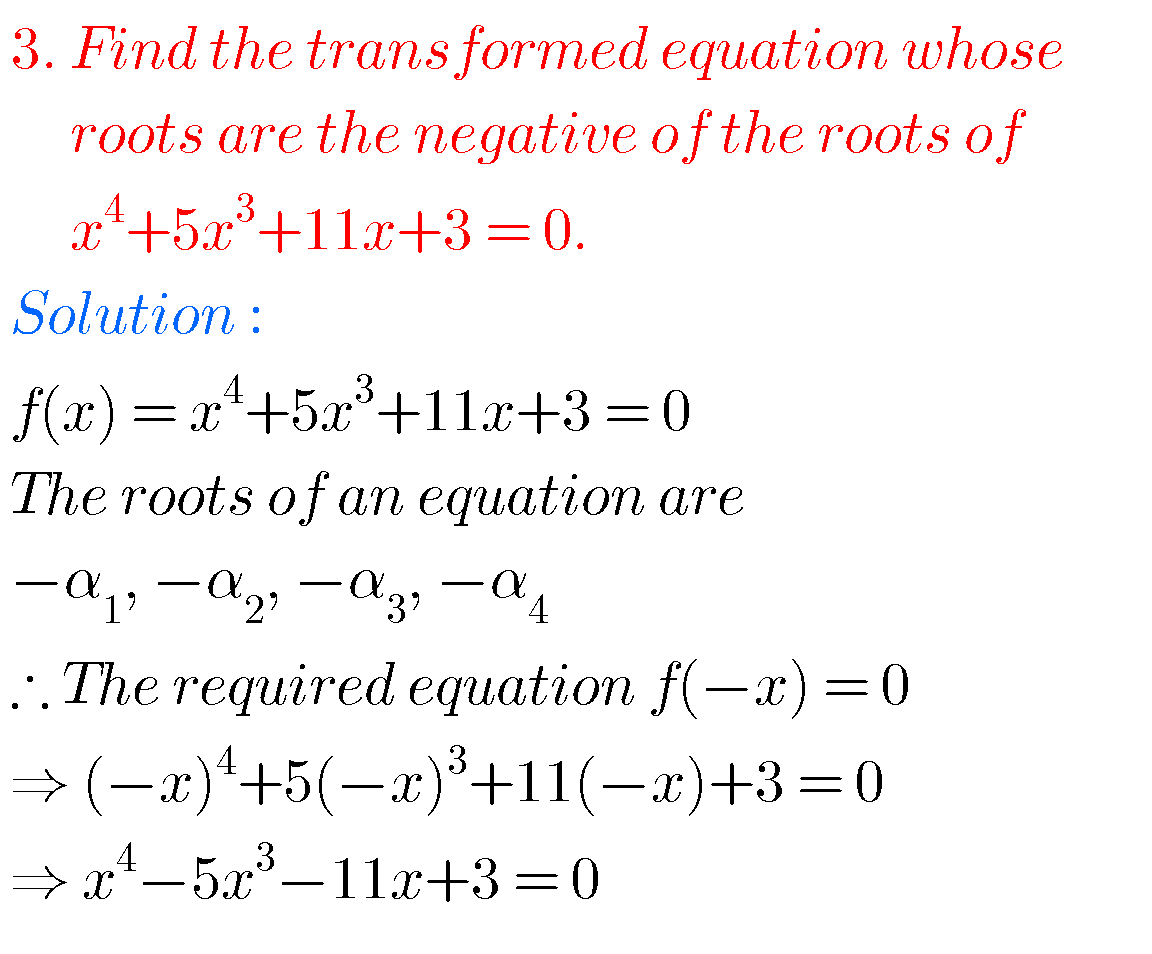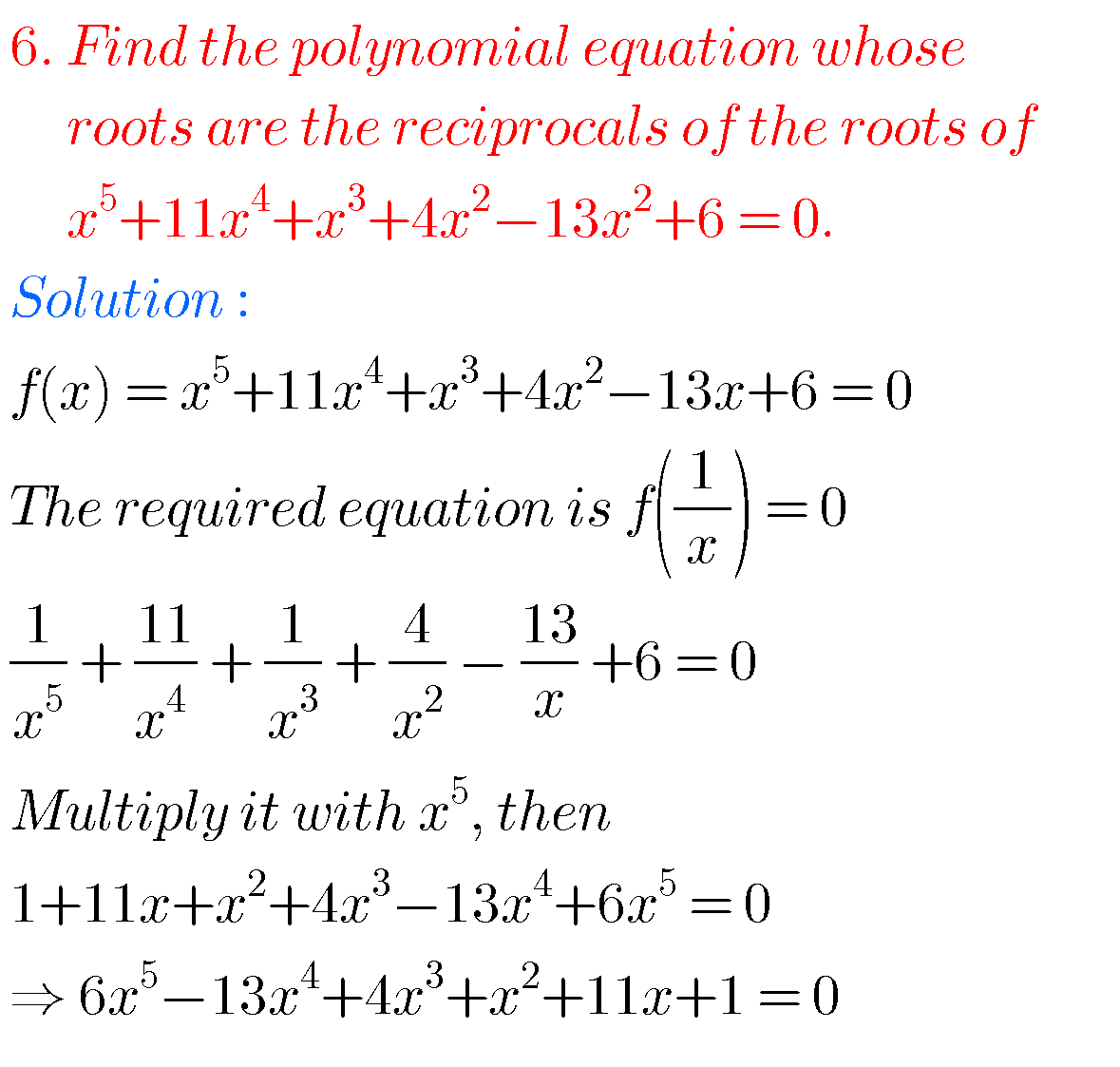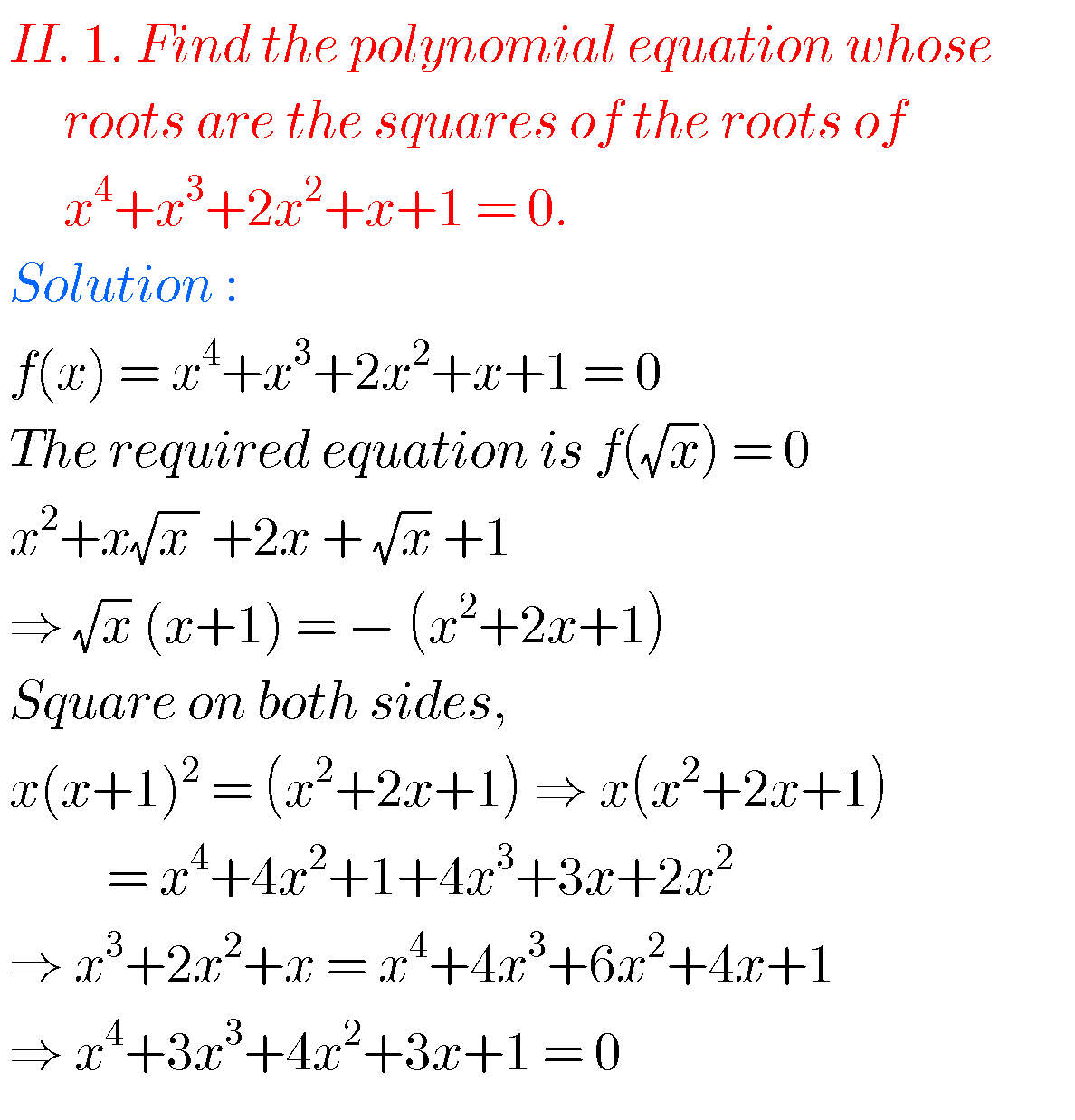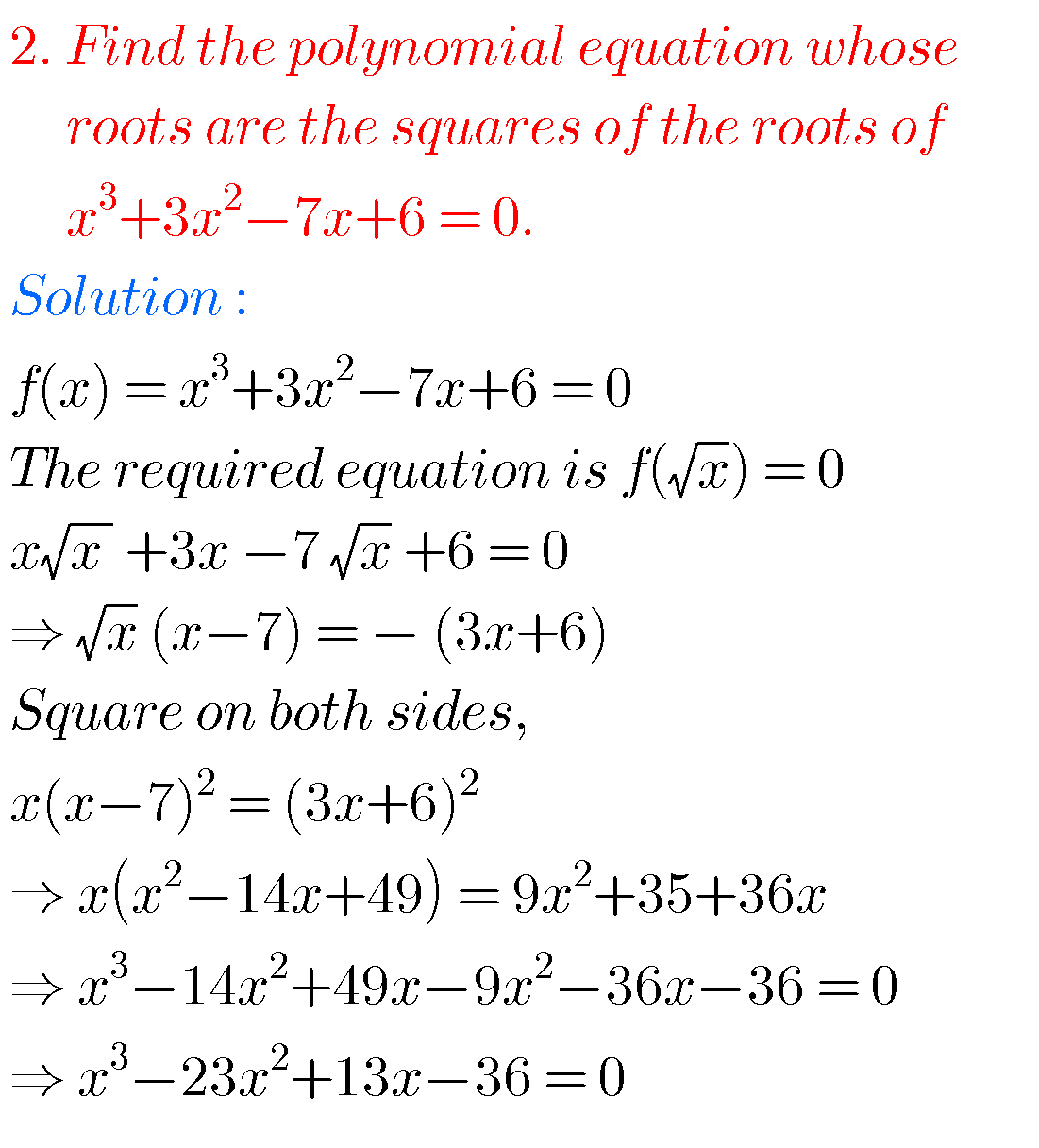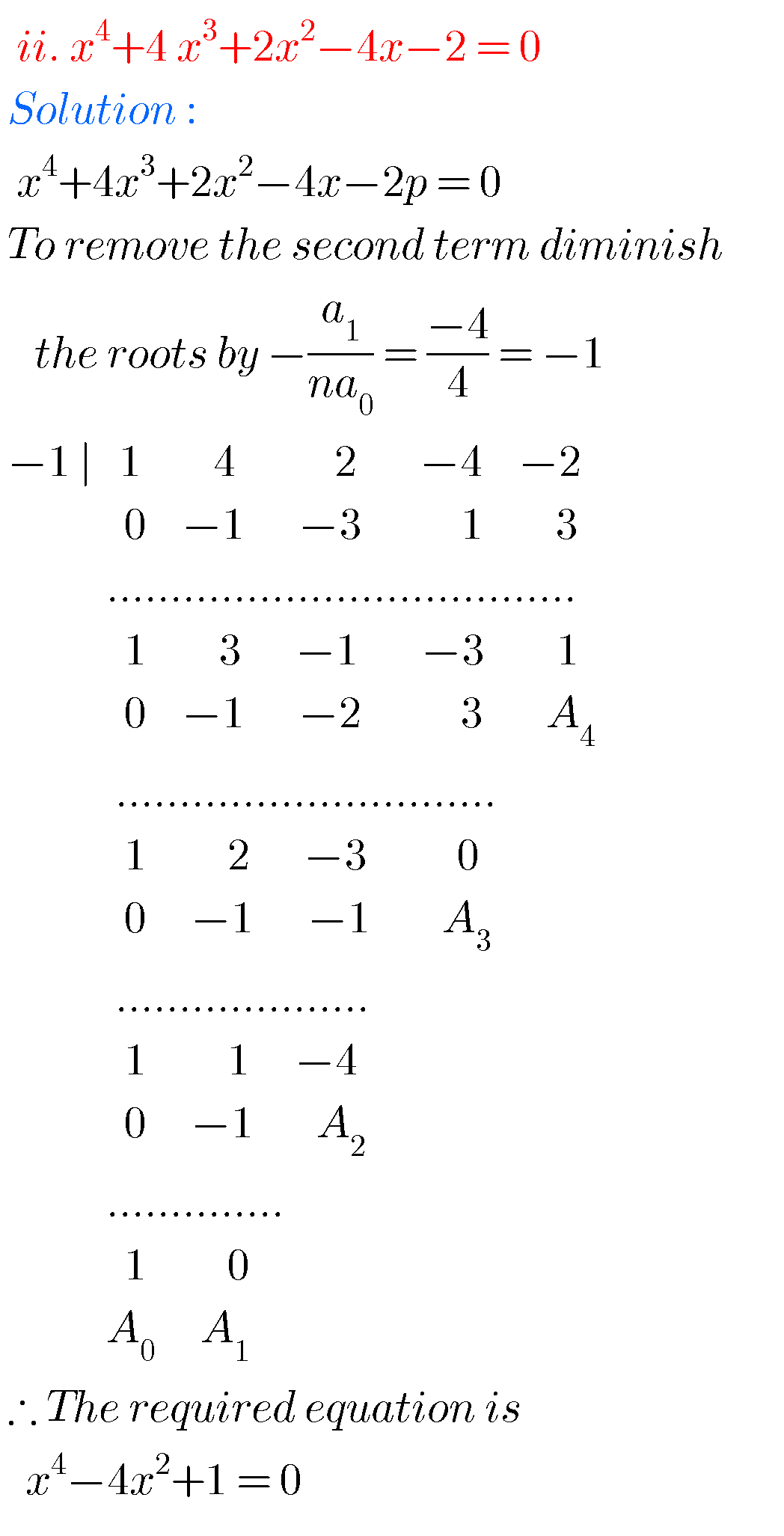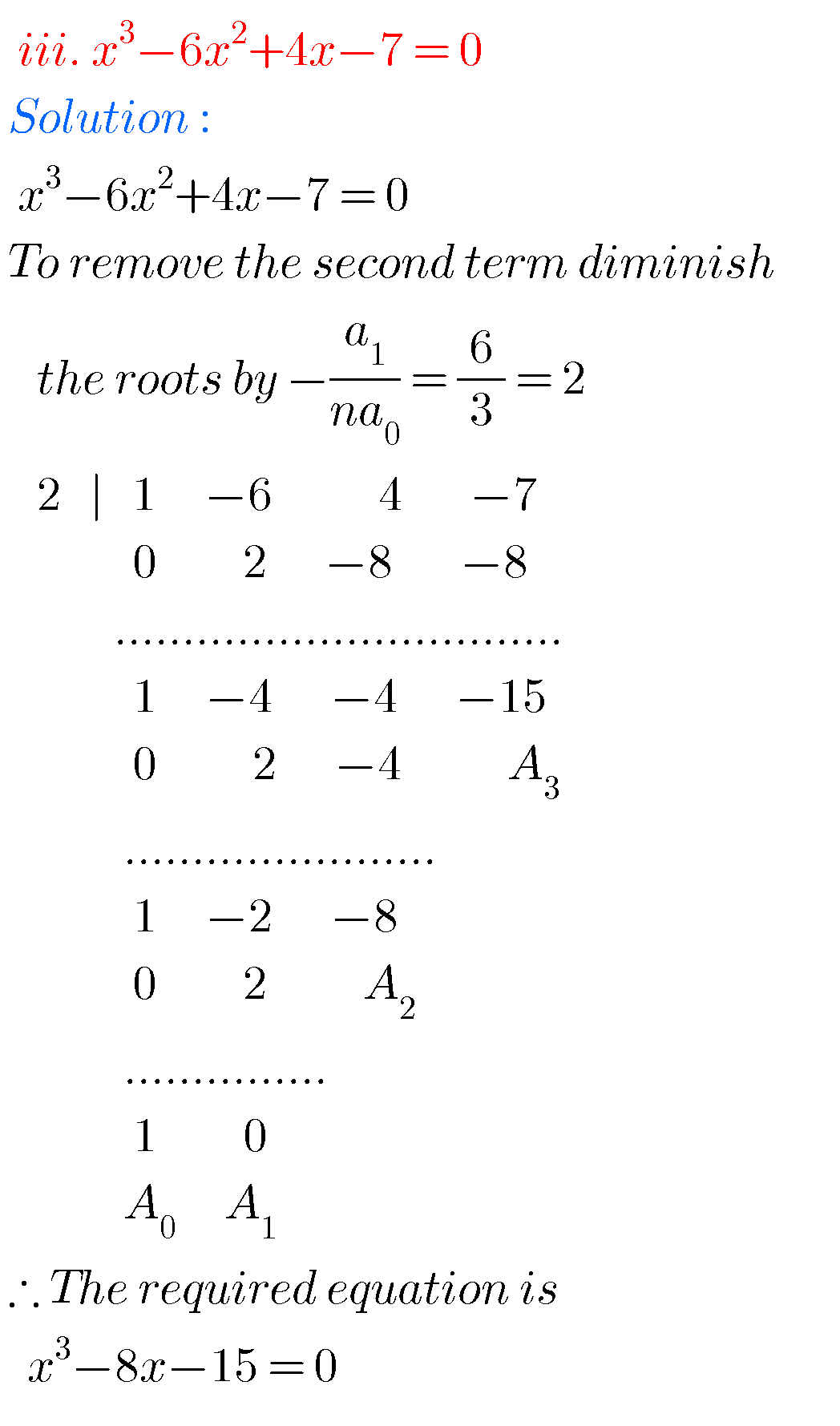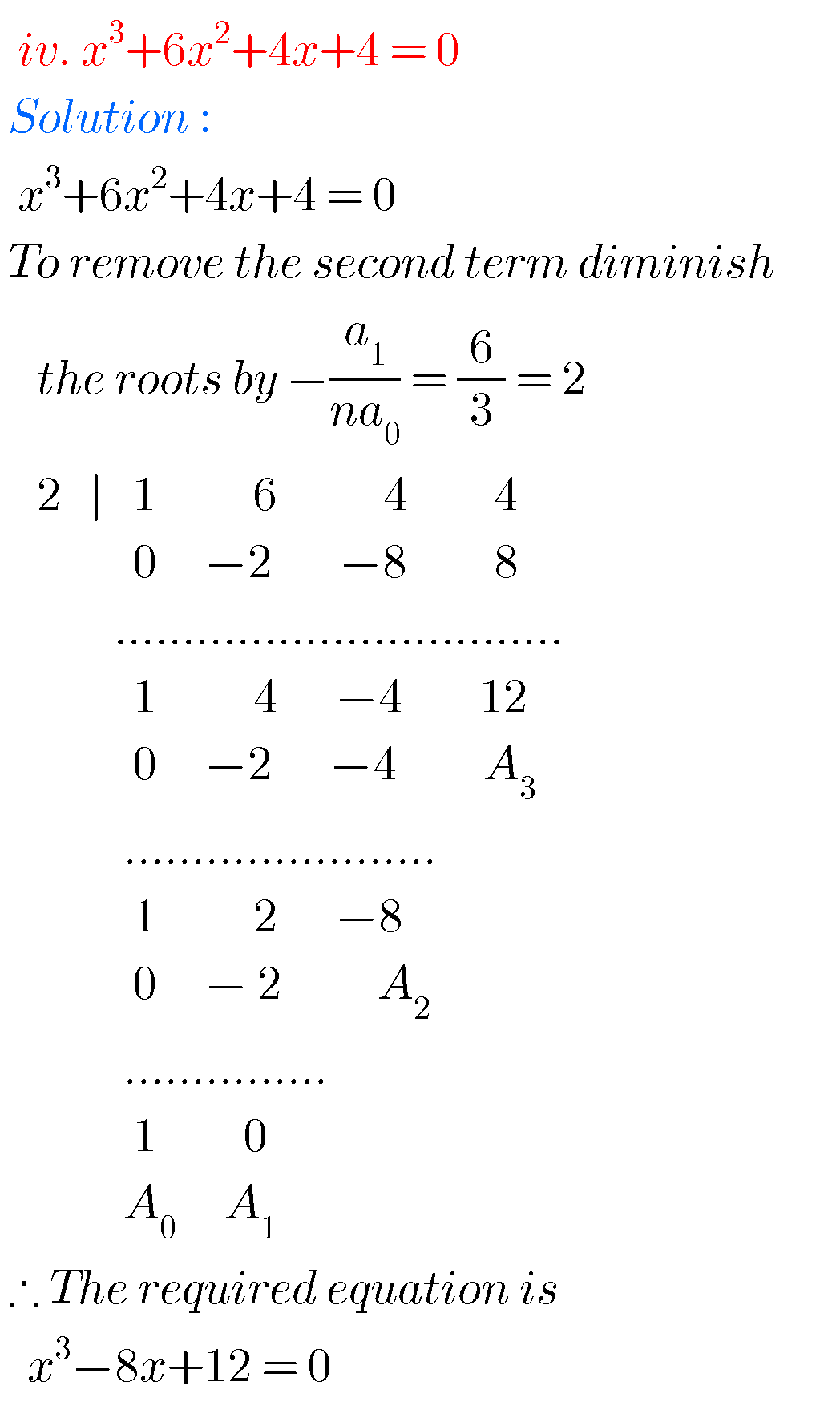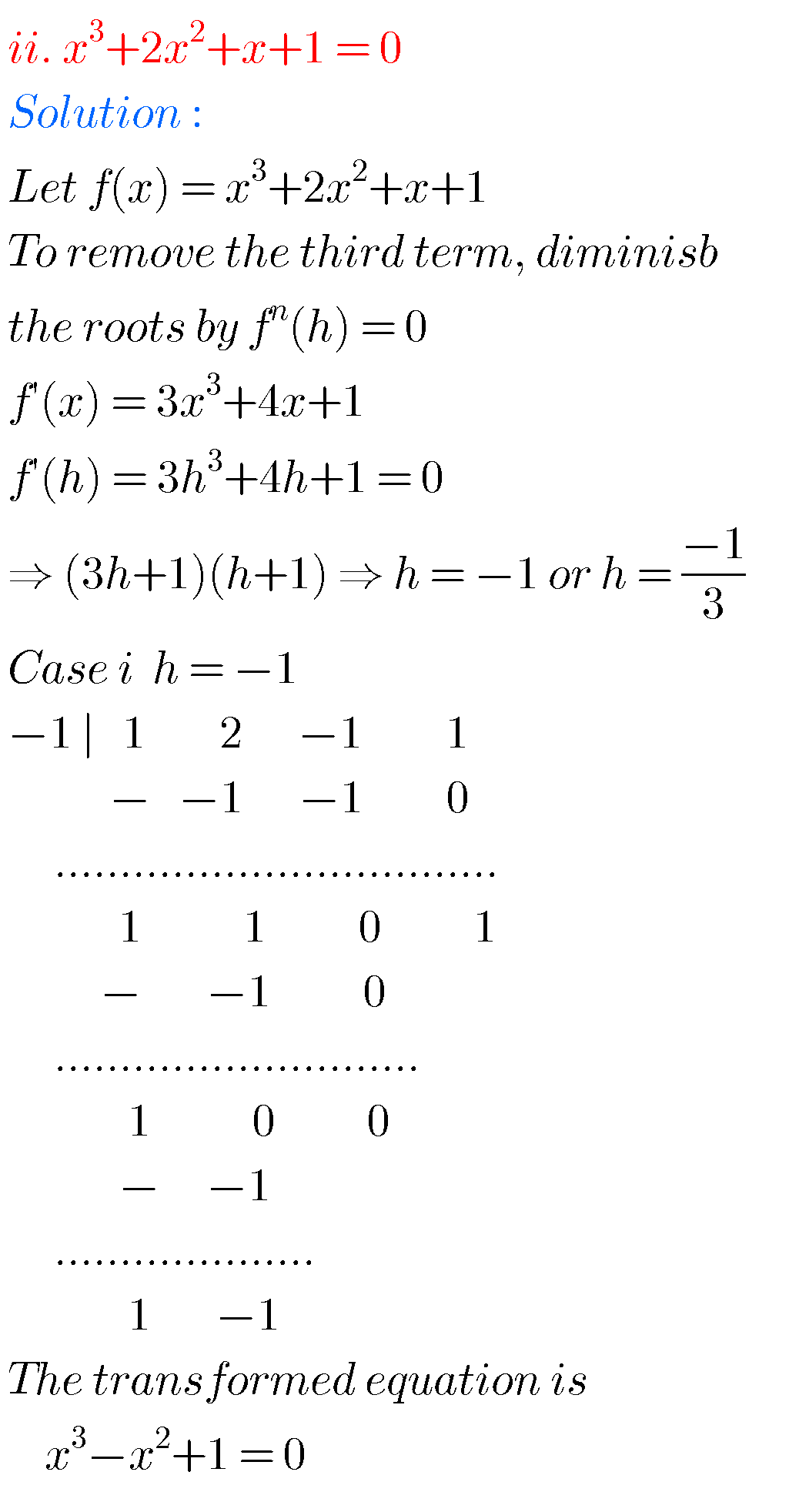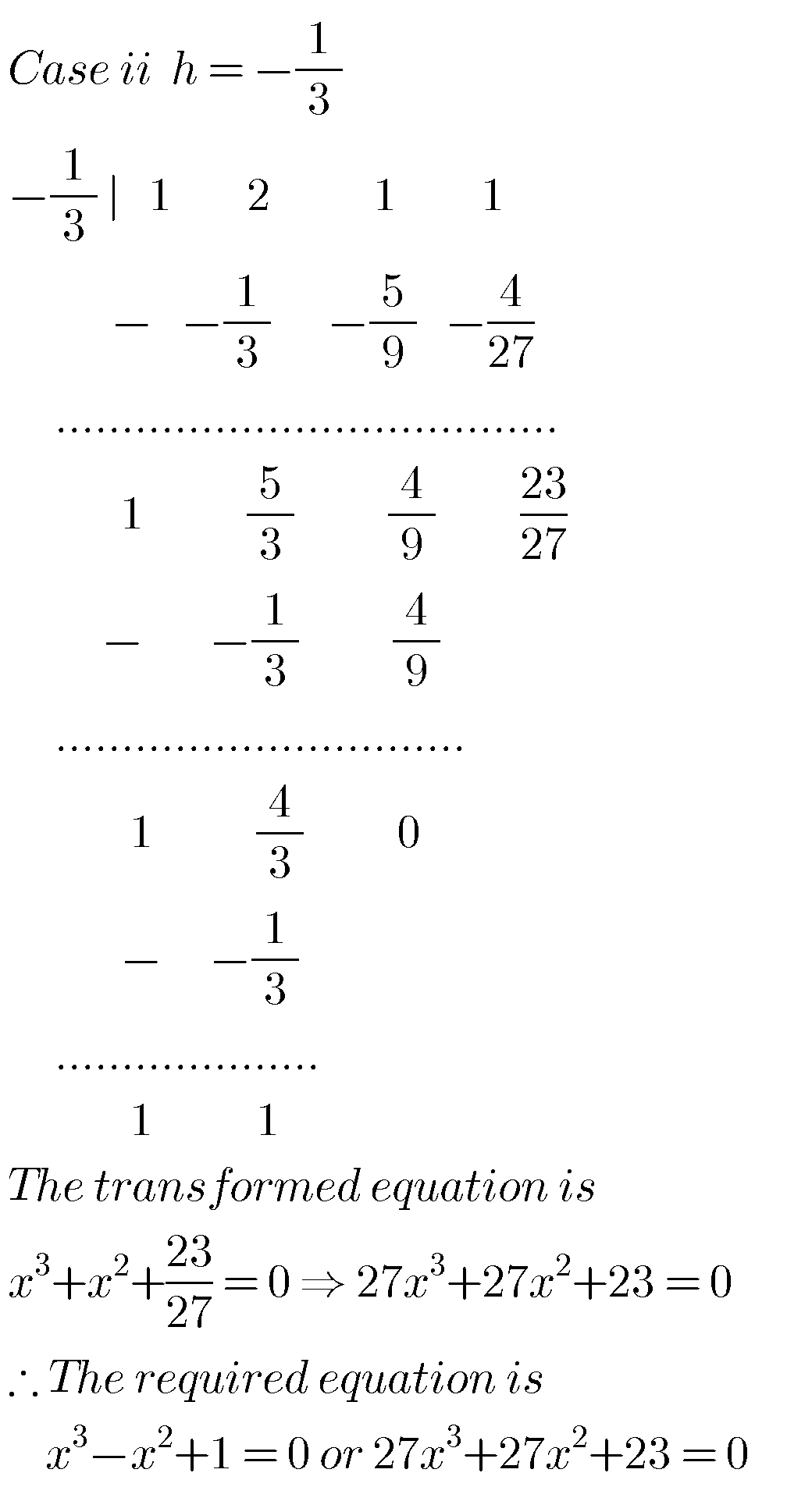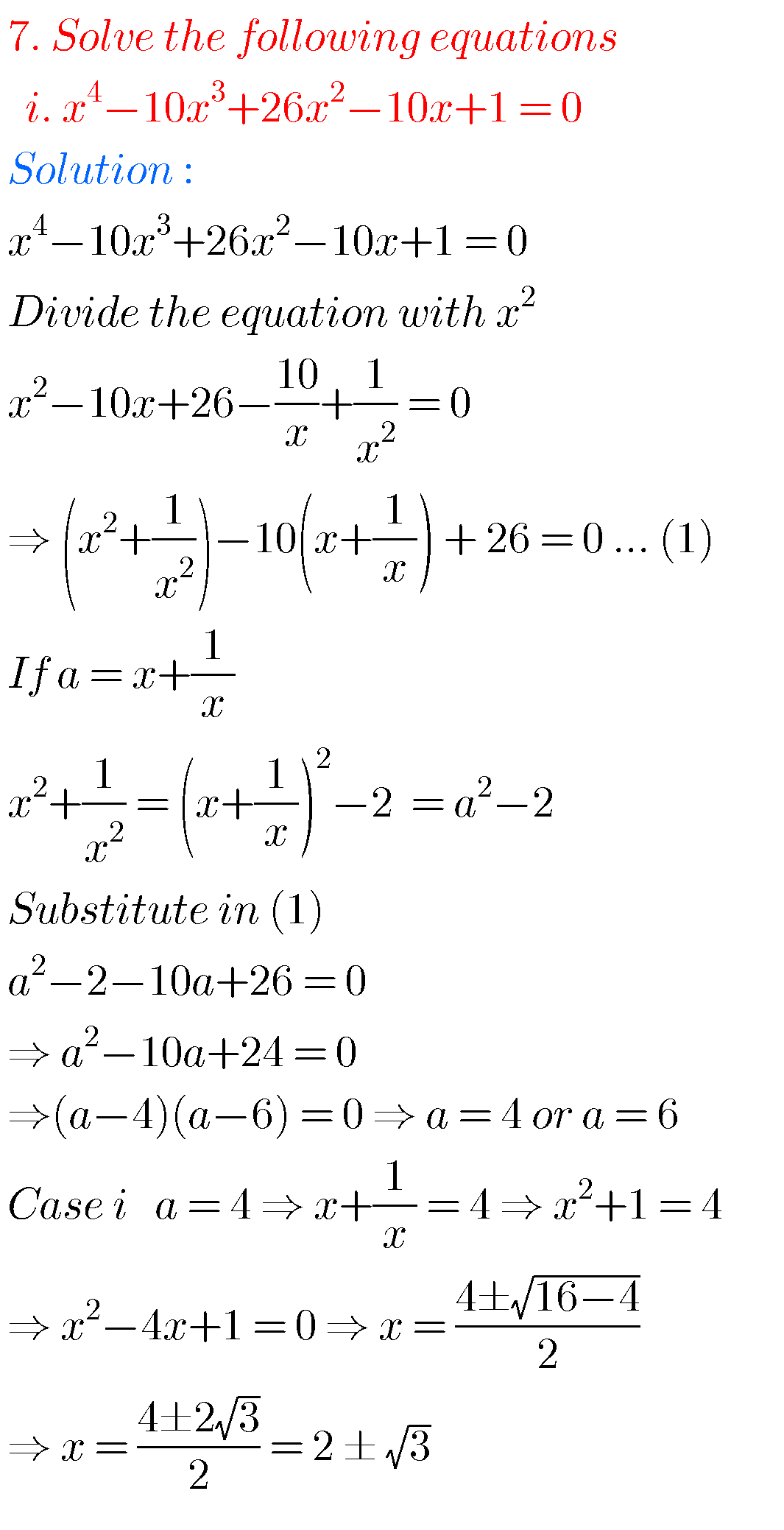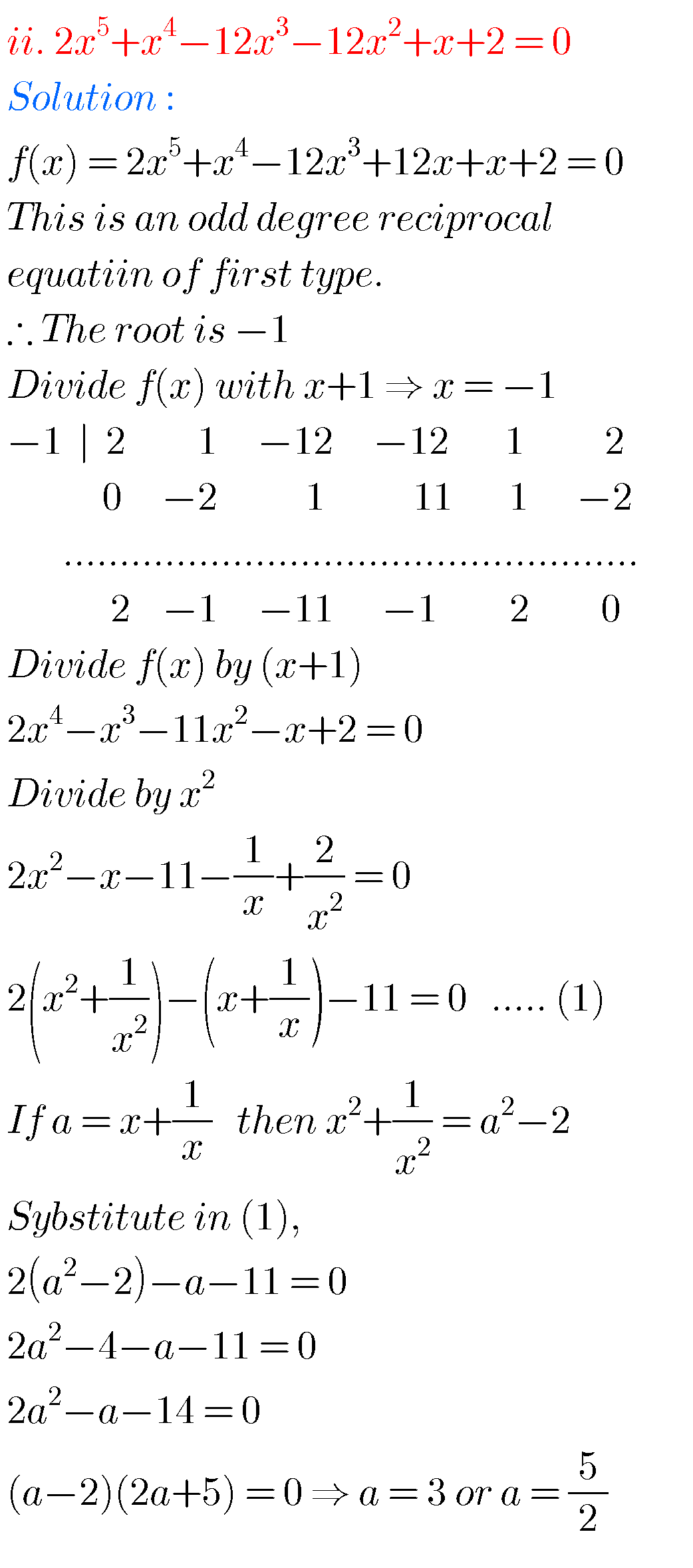Note : Observe the solutions and try them in your own methods.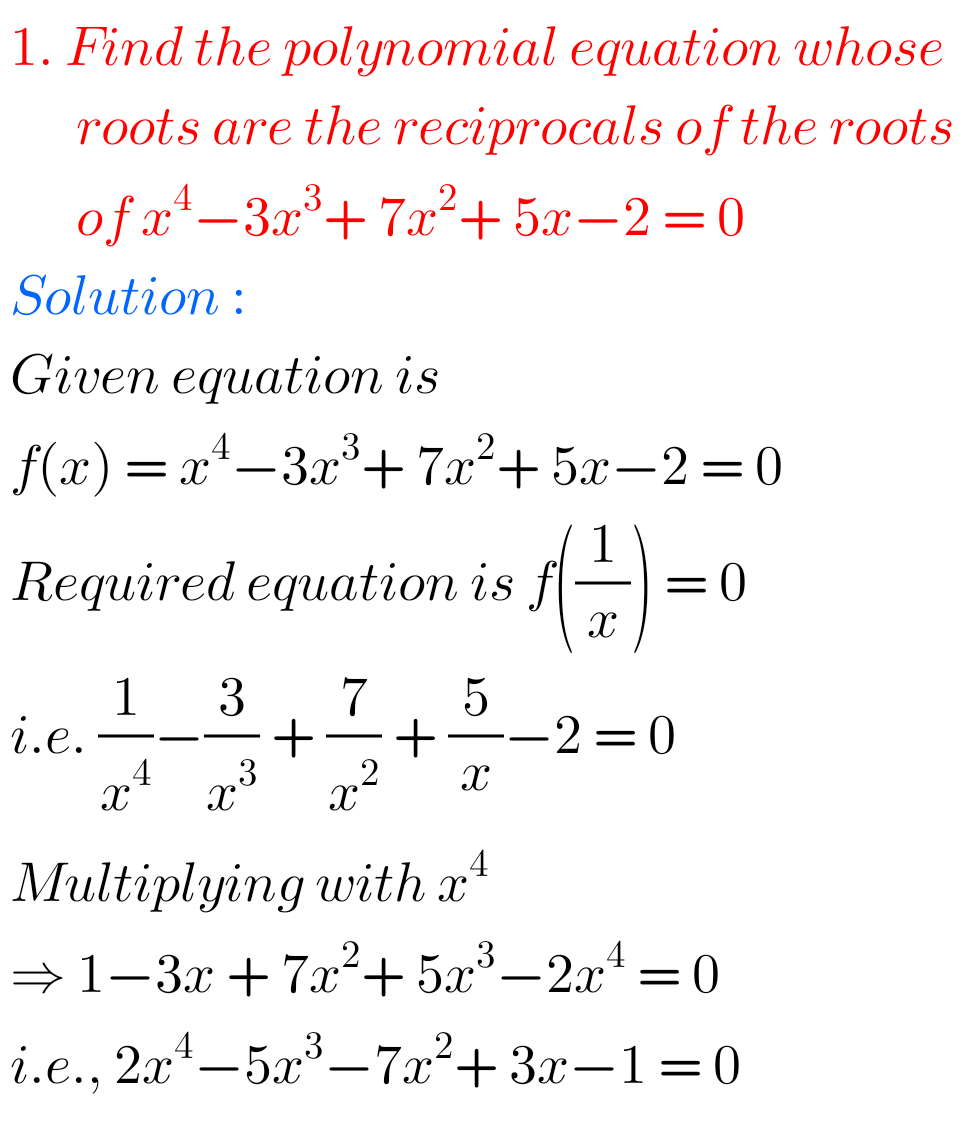Inter 1a exercise 6(a) solutions

Inter maths 1a exercise 6(b) solutions

class 10 maths polynomials solutions

Ncert maths class 8 exponents and powers

Ncert maths class 7 fractions and decimals

Ncert maths class 6 playing with numbers

### 1 thought on “Inter maths solutions for Theory of Equations,exercise 4(a),4(b),4(c),4(d) chapter 4 Intermediate maths IIA”

1.Thank you